# Consistent coupling of positions and rotations for embedding 1D Cosserat beams into 3D solid volumes

## Abstract

The present article proposes a mortar-type finite element formulation for consistently embedding curved, slender beams into 3D solid volumes. Following the fundamental kinematic assumption of undeformable cross-section s, the beams are identified as 1D Cosserat continua with pointwise six (translational and rotational) degrees of freedom describing the cross-section (centroid) position and orientation. A consistent 1D-3D coupling scheme for this problem type is proposed, requiring to enforce both positional and rotational constraints. Since Boltzmann continua exhibit no inherent rotational degrees of freedom, suitable definitions of orthonormal triads are investigated that are representative for the orientation of material directions within the 3D solid. While the rotation tensor defined by the polar decomposition of the deformation gradient appears as a natural choice and will even be demonstrated to represent these material directions in a $$L_2$$-optimal manner, several alternative triad definitions are investigated. Such alternatives potentially allow for a more efficient numerical evaluation. Moreover, objective (i.e. frame-invariant) rotational coupling constraints between beam and solid orientations are formulated and enforced in a variationally consistent manner based on either a penalty potential or a Lagrange multiplier potential. Eventually, finite element discretization of the solid domain, the embedded beams, which are modeled on basis of the geometrically exact beam theory, and the Lagrange multiplier field associated with the coupling constraints results in an embedded mortar-type formulation for rotational and translational constraint enforcement denoted as full beam-to-solid volume coupling (BTS-FULL) scheme. Based on elementary numerical test cases, it is demonstrated that a consistent spatial convergence behavior can be achieved and potential locking effects can be avoided, if the proposed BTS-FULL scheme is combined with a suitable solid triad definition. Eventually, real-life engineering applications are considered to illustrate the importance of consistently coupling both translational and rotational degrees of freedom as well as the upscaling potential of the proposed formulation. This allows the investigation of complex mechanical systems such as fiber-reinforced composite materials, containing a large number of curved, slender fibers with arbitrary orientation embedded in a matrix material.

## Introduction

Embedding fibers or beams, i.e. solid bodies that can mechanically be modeled as dimensionally reduced 1D structures since one spatial dimension is much larger than the other two, into a 3D matrix material is a common approach to enhance the mechanical properties of a structure. Fiber-reinforced structures can be found in many different fields, e.g. in form of steel reinforcements within concrete structures, lightweight fiber-reinforced composite materials based on carbon, glass or polymer fibers in a plastic matrix, or additively manufactured components allowing for a very flexible and locally controlled reinforcement of plastic, metal, ceramic or concrete matrix materials [32, 33, 42]. At a different length scale, fiber embeddings play a key role for essential processes in countless biological systems, e.g. in the form of embedded networks (e.g. cytoskeleton, extracellular matrix, mucus) or bundles (e.g. muscle, tendon, ligament) [2, 21, 31, 41]. Most of these applications are characterized by geometrically complex embeddings of arbitrarily oriented, slender and potentially curved fibers. Computational models predicting the response of such reinforced structures are essential for a time- and cost-efficient design and development of technical products, but also to gain fundamental understanding of biological systems at length scales that are not accessible via experiments. In the context of computational modeling, as considered in the following, the embedded 1D structures will be referred to as fibers or beams, respectively, and the 3D matrix as solid.

One common modeling approach for this physical beam-to-solid volume coupling problem is based on homogenized, anisotropic material models for the combined fiber-matrix structure [1, 70]. This widely used approach is appealing since, e.g. no additional degrees of freedom are required to model individual fibers, and existing simulation tools can be used as long as they support anisotropic material laws. However, such models cannot give detailed information about the interactions between fibers and surrounding matrix as, e.g. required to study mechanisms of failure. Moreover, the fiber distribution in the solid has to be sufficiently homogeneous and a separation of scales is required, i.e. the fiber size has to be sufficiently small as compared to the smallest dimension of the overall structure. Eventually, when modeling new fiber arrangements, the homogenization step inherent to these continuum models requires sub-scale information, e.g. provided by a model with resolved fiber geometries.

Another modeling approach consists of fully describing the fibers and surrounding solid material as 3D continua. This leads to a surface-to-surface coupling problem at the 2D interface between fiber surface and surrounding solid. In the context of the finite element method, these surfaces can be tied together by either applying fiber and solid discretizations that are conforming at the shared interface or via interface coupling schemes accounting for non-matching meshes, such as the mortar method [45, 47,48,49]. Alternatively, extended finite element methods (XFEM)  or immersed finite element methods [29, 55] can be used to represent 2D fiber surfaces embedded in an entirely independent background solid mesh. While such fully resolved modeling approaches allow to study local effects with high spatial resolution, the significant computational effort associated with these models prohibits their usage for large-scale systems with a large number of slender fibers.

The class of applications considered here typically involves very slender fibers. In this regime it is well justified, and highly efficient from a computational point of view, to model individual fibers as beams, e.g. based on the geometrically exact beam theory [9, 12, 14, 24, 30, 36, 38, 51, 52, 59,60,61], which is known to combine high model accuracy and computational efficiency [5, 53]. Based on the fundamental kinematic assumption of undeformable cross-section s, such beam models can be identified as 1D Cosserat continua with six degrees of freedom defined at each centerline point to describe the cross-section position (three translational degrees of freedom) and orientation (three rotational degrees of freedom). Thus, the problem of beams embedded in a 3D solid volume can be classified as mixed-dimensional 1D-3D coupling problem between 1D Cosserat continua and a 3D Boltzmann continuum. A variety of 1D-3D coupling approaches exist in the literature, however most of them involve truss/string models, i.e. 1D structural models account only for internal elastic energy contributions from axial tension, e.g. [13, 17, 20, 25, 26, 43, 50]. Work on the 1D-3D coupling between beams, i.e. full Cosserat continua, and solids is much rarer. In , collocation along the beam centerline is applied to couple beams with a surrounding solid material. A mortar-type coupling approach is proposed in the authors’ previous work , where a Lagrange multiplier field is defined along the beam centerline to weakly enforce the coupling constraints. The 1D-3D coupling between beams and a surrounding fluid field, as relevant for fluid-structure-interaction (FSI) problems, has been considered in some recent contributions [22, 68].

All the aforementioned 1D-3D beam-to-solid coupling schemes have in common that only the beam centerline positions, but not the cross-section orientations, are coupled to the solid, which will be denoted as translational 1D-3D coupling. In such models, an embedded fiber can still perform local twist/torsional rotations, i.e. cross-section rotations with respect to its centerline tangent vector, relative to the solid. While this simplified coupling procedure can reasonably describe the mechanics of certain problem classes where such relative rotations will rarely influence the global system response, e.g. embedding of straight fibers with circular cross-section shape, for most practical applications a more realistic description of the physical problem requires to also couple the rotations of beam and solid.

In a very recent approach by  the full 1D-3D coupling problem involving positions and rotations has been addressed for the first time. The coupling of the two directors spanning the (undeformable) beam cross-section with the underlying solid continuum together with the coupling of the cross-section centroids results in a total of nine coupling constraints. One specific focus of this interesting contribution lies on a static condensation strategy, which allows to eliminate the associated Lagrange multipliers and the beam balance equations from the final, discrete system of equations. The requirement of a $$C^1$$-continuous spatial discretization of the solid domain, as resulting from the proposed condensation strategy, is satisfied by employing NURBS-based test and trial functions.

The present work proposes a full 1D-3D coupling approach based on only six, i.e. three translational and three rotational, coupling constraints between the cross-section s of 1D beams, modeled according to the geometrically exact beam theory, and a 3D solid. The finite element method is employed for spatial discretization of all relevant fields. Consistently deriving the full 1D-3D coupling on the beam centerline from a 2D-3D coupling formulation on the beam surface via a first-order Taylor series expansion of the solid displacement field would require to fully couple the two orthonormal directors spanning the (undeformable) beam cross-section with the (in-plane projection of the) solid deformation gradient evaluated at the cross-section centroid position. It is demonstrated that such an approach, which suppresses all in-plane deformation modes of the solid at the coupling point, might result in severe locking effects in the practically relevant regime of coarse solid mesh sizes. Therefore, as main scientific contribution of this work, different definitions of orthonormal triads are proposed that are representative for the orientation of material directions of the 3D continuum in an average sense, without additionally constraining in-plane deformation modes when coupled to the beam cross-section. It is shown that the rotation tensor defined by the polar decomposition of the (in-plane projection of the) deformation gradient appears as a natural choice for this purpose, which even represents the average orientation of material directions of the 3D continuum in a $$L_2$$-optimal manner. Moreover, several alternative solid triad definitions are investigated that potentially allow for a more efficient numerical evaluation.

Once these solid triads have been defined, objective (i.e. frame-invariant) rotational coupling constraints in the form of relative rotations are formulated for each pair of triads representing the beam and solid orientation. Their variationally consistent enforcement either based on a penalty potential or a Lagrange multiplier potential, with an associated Lagrange multiplier field representing a distributed coupling moment along the beam centerline, is shown. Eventually, finite element discretization of the Lagrange multiplier and relative rotation vector field along the beam centerline results in an embedded mortar-type formulation for rotational constraint enforcement. In combination with a previously developed mortar-type formulation (BTS-TRANS) for translational 1D-3D coupling , this results in a full 1D-3D coupling approach denoted as full beam-to-solid volume coupling scheme (BTS-FULL). Finite element discretization of the solid and the embedded (potentially curved) beams inevitably results in non-matching meshes, which underlines the importance of a consistently embedded mortar-type formulation as proposed in this work. Based on elementary numerical test cases, it is demonstrated that a consistent spatial convergence behavior can be achieved and potential locking effects can be avoided if the proposed BTS-FULL scheme is combined with a suitable solid triad definition. Eventually, real-life engineering applications are considered to illustrate the importance of consistently coupling both translational and rotational degrees of freedom as well as the upscaling potential of the proposed formulation to study complex mechanical systems such as fiber-reinforced composite materials, containing a large number of curved, slender fibers with arbitrary orientation embedded in a matrix material.

The remainder of this work is organized as follows: In Sect. 2, we state the fundamental modeling assumptions of the proposed BTS-FULL scheme. Specifically, the importance of enforcing both rotational and translational coupling conditions is demonstrated, and the general implications of a 1D-3D coupling approach are discussed. In Sect. 3, we give a short summary of the theory of large rotations as required to formulate rotational coupling conditions. In Sect. 4, the governing equations for the solid and beam domains are presented, and objective rotational coupling constraints are defined and enforced in a variationally consistent manner, either based on a penalty or a Lagrange multiplier potential. In Sect. 5, we propose different definitions of orthonormal triads that are suitable to represent the orientation of solid material directions in an average sense. In Sect. 6, discretization of the coupling conditions based on the finite element method is considered, once in a Gauss point-to-segment manner and once as mortar-type approach along with a weighted penalty regularization. Finally, numerical examples, carefully selected to verify different aspects of the proposed formulation, are presented in Sect. 7.

## Motivation and modeling assumptions

In Sect. 2.1, the main modeling assumptions generally underlying 1D-3D coupling schemes will be discussed. Subsequently, in Sect. 2.2, the importance of a full position and rotation coupling (BTS-FULL) will be motivated for general application scenarios, and special cases will be discussed, where also a purely translational coupling (BTS-TRANS) can be considered as reasonable approximation.

### Modeling assumptions underlying the 1D-3D coupling

The considered class of 1D-3D coupling schemes is based on the assumption that the fiber material is stiff compared to the solid material, and local fiber cross-section dimensions are small compared to the global solid dimensions. Thus, the solid may be discretized without subtracting the fiber volume, formally resulting in overlapping solid and fiber domains. While consistent 2D-3D coupling on the fiber surface would allow for high-resolution stress field predictions in the direct vicinity of the 2D fiber-solid interface, such approaches require an evaluation of coupling constraints on a 2D interface and a sufficient discretization resolution of the solid with mesh sizes smaller than the fiber cross-section dimensions, thus in large parts deteriorating the advantages provided by a reduced dimensional description of the fibers.

In truly 1D-3D coupling approaches, the coupling conditions are exclusively defined along the beam centerline, thus preserving the computational advantages of the dimensionally reduced beam models. Of course, such approaches inevitably introduce a modeling error as compared to the 2D-3D coupling, i.e. the surface tractions on the 2D beam-solid interface are approximated by localized resultant line forces and moments acting on the beam centerline. This has a significant impact on the analytical solution of the problem, as line loads acting on a 3D continuum result in singular stress and displacement fields, cf. [19, 44, 66]. Thus, convergence of the 1D-3D solution towards the 2D-3D solution is not expected. However, in the realm of the envisioned applications, we are rather interested in global system responses than in local stress distributions in the direct vicinity of the fibers. Thus, practically relevant solid element sizes are considered that are larger than the fiber cross-section dimensions. In this regime of mesh resolutions, this inherent modeling error of 1D-3D approaches can typically be neglected.

To verify this statement, consider a plane problem of a beam cross-section, loaded with a moment, that is coupled to a solid finite element as depicted in Fig. 1. As long as the cross-section diameter is smaller than the solid finite element mesh size, the resulting discrete nodal forces $$F_S$$ acting on the solid are independent of the employed coupling approach, i.e. either 1D-3D coupling with associated coupling moment M (Fig. 1, left) or 2D-3D coupling with associated coupling surface load $$\tau$$ (Fig. 1, right). Obviously, this is an idealized setting, but it illustrates that 1D-3D coupling approaches can be considered as valid models for solid mesh sizes larger than the cross-section diameter, which will also be verified in Sect. 7. For a more detailed discussion of this topic the interested reader is referred to our previous publication , specifically to Figure 15 in , which depicts an analogous scenario for the coupling of translational degrees of freedom.

### Motivation for full translational and rotational coupling

To differentiate the scope of validity of the proposed BTS-FULL scheme (coupling of positions and rotations) and of existing BTS-TRANS schemes (coupling of positions only), two application scenarios are discussed.

As first scenario, systems are considered (i) that contain only transversely isotropic fibers (e.g. circular cross-section shape and initially straight) and (ii) whose global system response is dominated by the axial and bending stiffness of the fibers, i.e. the torsional contribution is negligible. As demonstrated in , BTS-TRANS schemes can be considered as a reasonable mechanical model in this case, since local (twist/torsional) rotations of the fibers with respect to their straight axes will rarely influence the global system response. Torsion-free beam models  represent an elegant mechanical description of the fibers for such applications.

As second scenario, systems are considered that contain transversely anisotropic fibers (e.g. non-circular cross-section shape or initially curved). First, it is clear that twist rotations of the fiber cross-section s with respect to the centerline tangent (even if not possible in their simplest form as rigid body rotations) will change the global system response, since such fibers exhibit distinct directions of maximal/minimal bending stiffness or initial curvature. Second, due to the inherent two-way coupling of bending and torsion in initially curved beams , bending deformation will inevitably induce torsion in such application scenarios, i.e. the global system stiffness is approximated as too soft if these torsional rotations are not transferred to the matrix by a proper coupling scheme. Thus, a unique and consistent mechanical solution for this scenario can only be guaranteed by BTS-FULL schemes.

### Remark 2.1

In fact, both aforementioned application scenarios might lead to non-unique static solutions if neglecting the rotational coupling. However, for transversely isotropic fibers the non-uniqueness only occurs at the local fiber level, i.e. the twist orientation of the fibers is not uniquely defined, which does not influence the global system response. The locally non-unique fiber orientation is typically only an issue from a numerical point of view (e.g. linear solvers), and can be effectively circumvented by employing, e.g. torsion-free beam models not exhibiting the relevant rotational degrees of freedom. For transversely anisotropic fibers, such local twist rotations will change the global system response. This gives rise to non-unique static solutions on the global level and, thus, has significant implications from a physical point of view.

## Large rotations

This section gives a brief overview on the mathematical treatment of finite rotations as required for the formulation of rotational coupling constraints. For a more comprehesive treatment of this topic, the interested reader is referred to [8, 12, 24, 38, 52, 60]. Let us consider a rotation tensor

\begin{aligned} \underline{\varvec{{\varLambda }}}= [\begin{array}{l} \underline{\varvec{{g}}}_{1}, \underline{\varvec{{g}}}_{2}, \underline{\varvec{{g}}}_{3} \end{array}] \in SO^3, \end{aligned}
(1)

where $$SO^3$$ is the special orthogonal group and the base vectors $$\underline{\varvec{{g}}}_{i}$$ form an orthonormal triad, that maps the Cartesian basis vectors $$\underline{\varvec{{e}}}_{i}$$ onto $$\underline{\varvec{{g}}}_{i}$$. In the following, a rotation pseudo-vector $$\underline{\varvec{{\psi }}}$$ is used for its parametrization, i.e. $$\underline{\varvec{{\varLambda }}}= \underline{\varvec{{\varLambda }}}(\underline{\varvec{{\psi }}})$$. The rotation vector describes a rotation by an angle $$\psi = \left\| \underline{\varvec{{\psi }}}\right\|$$ around the rotation axis $$\underline{\varvec{{e}}}_{\psi }= \underline{\varvec{{\psi }}}/ \left\| \underline{\varvec{{\psi }}}\right\|$$. The parametrization can be given by the well-known Rodrigues formula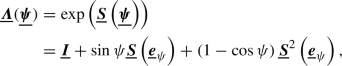(2)

where $$\exp (\cdot )$$ is the exponential map. Furthermore,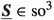is a skew-symmetric tensor, where $$\hbox {so}^3$$ represents the set of skew-symmetric tensors with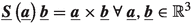. The inverse of the Rodrigues formula (2), i.e. the rotation vector as a function of the rotation tensor, will be denoted as $$\underline{\varvec{{\psi }}}(\underline{\varvec{{\varLambda }}}) = {{\,\mathrm{rv}\,}}(\underline{\varvec{{\varLambda }}})$$ in the remainder of this work. In practice, Spurrier’s algorithm  can be used for the extraction of the rotation vector.

Two triads $$\underline{\varvec{{\varLambda }}}_1(\underline{\varvec{{\psi }}}_{1})$$ and $$\underline{\varvec{{\varLambda }}}_2(\underline{\varvec{{\psi }}}_{2})$$, with their respective rotation vectors $$\underline{\varvec{{\psi }}}_{1}$$ and $$\underline{\varvec{{\psi }}}_{2}$$, can be related by the relative rotation $$\underline{\varvec{{\varLambda }}}_{21}(\underline{\varvec{{\psi }}}_{21})$$. The relative rotation is given by

\begin{aligned} \begin{aligned} \underline{\varvec{{\varLambda }}}_{2}(\underline{\varvec{{\psi }}}_{2})&= \underline{\varvec{{\varLambda }}}_{21}(\underline{\varvec{{\psi }}}_{21}) \underline{\varvec{{\varLambda }}}_1(\underline{\varvec{{\psi }}}_{1})\\&\Updownarrow \\ \underline{\varvec{{\varLambda }}}_{21}(\underline{\varvec{{\psi }}}_{21})&= \underline{\varvec{{\varLambda }}}_2(\underline{\varvec{{\psi }}}_{2}) \underline{\varvec{{\varLambda }}}_1(\underline{\varvec{{\psi }}}_{1})^{\mathrm T}, \end{aligned} \end{aligned}
(3)

with the identity $$\underline{\varvec{{\varLambda }}}^{\mathrm T}=\underline{\varvec{{\varLambda }}}^{-1}$$ for all elements of $$SO^3$$. Thus, the (non-additive) rotation vector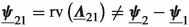describes the relative rotation between $$\underline{\varvec{{\varLambda }}}_1$$ and $$\underline{\varvec{{\varLambda }}}_2$$.

In a next step, the infinitesimal variations of the rotation tensor shall be considered, which can be expressed either by an infinitesimal additive variation $$\delta \underline{\varvec{{\psi }}}$$ of the rotation vector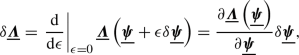(4)

or by a infinitesimal multiplicative rotation variation $$\delta \underline{\varvec{{\theta }}}$$, which is also denoted as spin vector: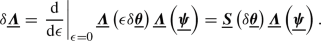(5)

While the definition of the multiplicative rotation variation (5) can often be found in the literature, e.g. in [14, 24, 61], the notation introduced for the additive rotation variation (4) simply represents the standard definition of partial differentiation, which is based on additive increments. With the relation above and the definition of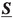, the variations of the triad basis vectors $$\delta \underline{\varvec{{g}}}_{i}$$ read

\begin{aligned} \delta \underline{\varvec{{g}}}_{i} = \delta \underline{\varvec{{\theta }}}\times \underline{\varvec{{g}}}_{i}. \end{aligned}
(6)

The infinitesimal additive and multiplicative rotation vector variations can be related according to

\begin{aligned} \delta \underline{\varvec{{\psi }}}= \underline{\varvec{{T}}}(\underline{\varvec{{\psi }}}) \delta \underline{\varvec{{\theta }}}, \end{aligned}
(7)

where the transformation matrix $$\underline{\varvec{{T}}}(\underline{\varvec{{\psi }}})$$  is defined as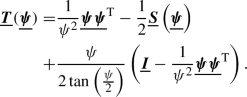(8)

In , the objective variation $$\delta _{o}$$ of a spatial quantity defined in a moving frame $$\underline{\varvec{{\varLambda }}}_1$$ is defined as the difference between the total variation and the variation of the base vectors of the moving frame. In the context of rotational coupling constraints this will be required when expressing the objective variation of a relative rotation vector $$\underline{\varvec{{\psi }}}_{21}$$:

\begin{aligned} \delta _{o}\underline{\varvec{{\psi }}}_{21} = \delta \underline{\varvec{{\psi }}}_{21} - \delta \underline{\varvec{{\theta }}}_1 \times \underline{\varvec{{\psi }}}_{21} = \underline{\varvec{{T}}}(\underline{\varvec{{\psi }}}_{21}) (\delta \underline{\varvec{{\theta }}}_{2} - \delta \underline{\varvec{{\theta }}}_{1}). \end{aligned}
(9)

For a detailed derivation of this expression for the objective variation the interested reader is referred to .

### Remark 3.1

Via right-multiplication of (8) with the rotation vector $$\underline{\varvec{{\psi }}}$$ it can easily be shown that $$\underline{\varvec{{\psi }}}$$ is an eigenvector (with eigenvalue 1) of $$\underline{\varvec{{T}}}$$ and also of $$\underline{\varvec{{T}}}^{\mathrm T}$$, i.e. $$\underline{\varvec{{T}}}\underline{\varvec{{\psi }}}= \underline{\varvec{{\psi }}}$$ and $$\underline{\varvec{{T}}}^{\mathrm T}\underline{\varvec{{\psi }}}= \underline{\varvec{{\psi }}}$$. This property will be beneficial for derivations presented in subsequent sections. Every vector parallel to $$\underline{\varvec{{\psi }}}$$ is also an eigenvector of $$\underline{\varvec{{T}}}$$. This can be interpreted in a geometrical way: If the additive increment $$\delta \underline{\varvec{{\psi }}}$$ to a rotation vector $$\underline{\varvec{{\psi }}}$$ is parallel to the rotation vector, i.e. $$\delta \underline{\varvec{{\psi }}}= \delta \psi \underline{\varvec{{e}}}_{\psi }$$ and $$\underline{\varvec{{\psi }}}= \psi \underline{\varvec{{e}}}_{\psi }$$, the resulting compound rotation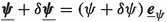is still defined around the rotation axis $$\underline{\varvec{{e}}}_{\psi }$$. In this case, the rotation increment is a plane rotation relative to $$\underline{\varvec{{\varLambda }}}(\underline{\varvec{{\psi }}})$$, and the multiplicative and additive rotational increments are equal to each other, $$\delta \underline{\varvec{{\psi }}}= \delta \underline{\varvec{{\theta }}}$$.

### Remark 3.2

In addition to $$\underline{\varvec{{\varLambda }}}$$, also the symbol $$\underline{\varvec{{R}}}$$ will be used in the following to represent rotation tensors.

## Problem formulation

We consider a 3D finite deformation full beam-to-solid volume coupling problem (BTS-FULL) as shown in Fig. 2. All quantities are refereed to a Cartesian frame $$\underline{\varvec{{e}}}_1, \underline{\varvec{{e}}}_2, \underline{\varvec{{e}}}_3$$. For simplicity, we focus on quasi-static problems in this work, while the presented BTS-FULL method is directly applicable to dynamic problems as well. The principle of virtual work serves as basis for the proposed finite element formulation. Contributions to the total virtual work of the system can be split into solid, beam and coupling terms, where the solid and beam terms are independent of the coupling constraints, i.e. well-established modeling and discretization techniques can be used for these single fields, cf. .

### Solid formulation

The solid body is modeled as a 3D Boltzmann continuum, defined by its domain $$\varOmega _{S,0}\subset {\mathbb {R}}^{3}$$ in the reference configuration, with boundary $$\partial \varOmega _{S,0}$$. Throughout this work, the subscript $$(\cdot )_0$$ indicates a quantity in the reference configuration. A solid material point can be identified by its reference position $$\underline{\varvec{{X}}}_S\in {\mathbb {R}}^{3}$$. The current position $$\underline{\varvec{{x}}}_S\in {\mathbb {R}}^{3}$$ relates to $$\underline{\varvec{{X}}}_S$$ via the displacement field $$\underline{\varvec{{u}}}_S\in {\mathbb {R}}^{3}$$, i.e.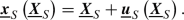(10)

The domain and surface of the solid in the deformed configuration are $$\varOmega _S$$ and $$\partial \varOmega _S$$, respectively. Virtual work contributions $$\delta W^S$$ of the solid are given by

\begin{aligned} \begin{aligned} \delta W^S=&\int _{\varOmega _{S,0}}{ \underline{\varvec{{S}}}: \delta \underline{\varvec{{E}}} \,\mathrm d V_0}\, \\&- \int _{\varOmega _{S,0}}{ \hat{\underline{\varvec{{b}}}}\cdot \delta \underline{\varvec{{u}}}_S \,\mathrm d V_0}\, - \int _{\varGamma _\sigma }{ \hat{\underline{\varvec{{t}}}}\cdot \delta \underline{\varvec{{u}}}_S \,\mathrm d A_0}\, , \end{aligned} \end{aligned}
(11)

where $$\delta$$ denotes the (total) variation of a quantity, $$\underline{\varvec{{S}}}\in {\mathbb {R}}^{3\times 3}$$ is the second Piola–Kirchhoff stress tensor, $$\underline{\varvec{{E}}}\in {\mathbb {R}}^{3\times 3}$$ is the work-conjugated Green–Lagrange strain tensor, $$\hat{\underline{\varvec{{b}}}}\in {\mathbb {R}}^{3}$$ is the body load vector and $$\hat{\underline{\varvec{{t}}}}\in {\mathbb {R}}^{3}$$ are surface tractions on the Neumann boundary $$\varGamma _\sigma \subset \partial \varOmega _{S,0}$$. The Green-Lagrange strain tensor is defined as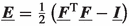, where the deformation gradient $$\underline{\varvec{{F}}}\in {\mathbb {R}}^{3\times 3}$$ is defined according to

\begin{aligned} \underline{\varvec{{F}}}= \frac{\partial \underline{\varvec{{x}}}_S}{\partial \underline{\varvec{{X}}}_S}. \end{aligned}
(12)

For the compressible or nearly incompressible solid material, we assume existence of a hyperelastic strain energy function $$\varPsi (\underline{\varvec{{E}}})$$, which allows to determine the second Piola–Kirchhoff stress tensor according to $$\underline{\varvec{{S}}}=\frac{\partial \varPsi (\underline{\varvec{{E}}})}{\partial \underline{\varvec{{E}}}}$$.

### Geometrically exact beam theory

The beams are modeled as 1D Cosserat continua embedded in 3D space based on the geometrically exact Simo–Reissner beam theory. Thus, each beam cross-section is described by six degrees of freedom, namely three positional and three rotational degrees of freedom. This results in six deformation modes of the beam: axial tension, bending ($$2\times$$), shear ($$2\times$$) and torsion.

The cross-section centroids are connected by the centerline curve $$\underline{\varvec{{r}}}(s) \in {\mathbb {R}}^{3}$$, where $$s\in [0,L] =: \varOmega _{B,0}\subset {\mathbb {R}}^{}$$ is the arc-length coordinate along the beam centerline $$\varOmega _{B,0}$$ in the reference configuration, and L the corresponding reference length. The displacement of the beam centerline $$\underline{\varvec{{u}}}_B(s)\in {\mathbb {R}}^{3}$$ relates the reference position $$\underline{\varvec{{r}}}_0$$ to the current position $$\underline{\varvec{{r}}}$$ via

\begin{aligned} \underline{\varvec{{r}}}(s) = \underline{\varvec{{r}}}_0(s) + \underline{\varvec{{u}}}_B(s). \end{aligned}
(13)

The orientation of the beam cross-section field is described by the following field of right-handed orthonormal triads $$\underline{\varvec{{\varLambda }}}_{B}(s) := [\underline{\varvec{{g}}}_{B1}(s), \underline{\varvec{{g}}}_{B2}(s), \underline{\varvec{{g}}}_{B3}(s)]=\underline{\varvec{{\varLambda }}}_{B}(\underline{\varvec{{\psi }}}_{B}(s)) \in SO^3$$, which maps the global Cartesian basis vectors $$\underline{\varvec{{e}}}_i$$ onto the local cross-section basis vectors $$\underline{\varvec{{g}}}_{Bi}(s) = \underline{\varvec{{\varLambda }}}_{B}\underline{\varvec{{e}}}_i$$ for $$i=1,2,3$$. Therein, $$\underline{\varvec{{\psi }}}_{B}\in {\mathbb {R}}^{3}$$ is the rotation pseudo-vector chosen as parametrization for the triad. Moreover, the triad field in the reference configuration is denoted as $$\underline{\varvec{{\varLambda }}}_{B,0}(s) := [\underline{\varvec{{g}}}_{B1,0}(s), \underline{\varvec{{g}}}_{B2,0}(s), \underline{\varvec{{g}}}_{B3,0}(s)]=\underline{\varvec{{\varLambda }}}_{B,0}(\underline{\varvec{{\psi }}}_{B,0}(s))$$, and the relative rotation between the triads in reference and current configuration is denoted as $$\underline{\varvec{{R}}}_B:= \underline{\varvec{{\varLambda }}}_{B}\underline{\varvec{{\varLambda }}}_{B,0}^{\mathrm T}$$. According to the fundamental kinematic assumption of undeformable cross-section s, the position of an arbitrary material point within the beam cross-section either in the reference or in the current configuration can be expressed as follows:

\begin{aligned} \underline{\varvec{{X}}}_B(s, \alpha , \beta )&= \underline{\varvec{{r}}}_0(s) + \alpha \underline{\varvec{{g}}}_{B2,0}(s) + \beta \underline{\varvec{{g}}}_{B3,0}(s), \end{aligned}
(14)
\begin{aligned} \underline{\varvec{{x}}}_B(s, \alpha , \beta )&= \underline{\varvec{{r}}}(s) + \alpha \underline{\varvec{{g}}}_{B2}(s) + \beta \underline{\varvec{{g}}}_{B3}(s), \end{aligned}
(15)

where $$\alpha$$ and $$\beta$$ represent in-plane coordinates. Based on a hyperelastic stored-energy function according to

\begin{aligned} \begin{aligned} \varPi _{\text {int},B}&= \int _{\varOmega _{B,0}}{ {\tilde{\varPi }}_{\text {int},B} \,\mathrm d s}\, \\ \text {with} \quad {\tilde{\varPi }}_{\text {int},B}&=\frac{1}{2}(\underline{\varvec{{\varGamma }}}^{\mathrm T}\underline{\varvec{{C}}}_F\underline{\varvec{{\varGamma }}}+ \underline{\varvec{{\varOmega }}}^{\mathrm T}\underline{\varvec{{C}}}_M\underline{\varvec{{\varOmega }}}) \end{aligned} \end{aligned}
(16)

the material force stress resultants $$\underline{\varvec{{F}}}=\frac{\partial {\tilde{\varPi }}_{\text {int},B}}{\partial \underline{\varvec{{\varGamma }}}}$$ and moment stress resultants $$\underline{\varvec{{M}}}=\frac{\partial {\tilde{\varPi }}_{\text {int},B}}{\partial \underline{\varvec{{\varOmega }}}}$$ can be derived. Here, $$\underline{\varvec{{\varGamma }}}\in {\mathbb {R}}^{3}$$ is a material deformation measure representing axial tension and shear, $$\underline{\varvec{{\varOmega }}}\in {\mathbb {R}}^{3}$$ is a material deformation measure representing torsion and bending, and $$\underline{\varvec{{C}}}_F\in {\mathbb {R}}^{3\times 3}$$ and $$\underline{\varvec{{C}}}_M\in {\mathbb {R}}^{3\times 3}$$ are cross-section constitutive matrices. Eventually, the beam contributions to the weak form are given by

\begin{aligned} \delta W^B= \delta \varPi _{\text {int},B}+ \delta W^B_{\text {ext}}, \end{aligned}
(17)

with the virtual work $$\delta W^B_{\text {ext}}$$ of external forces and moments.

### Full beam-to-solid volume coupling (BTS-FULL)

In the proposed BTS-FULL method, the pointwise six degrees of freedom associated with the beam centerline positions and cross-section triads are coupled to the surrounding solid, i.e.

\begin{aligned} \underline{\varvec{{r}}}- \underline{\varvec{{x}}}_S&= \underline{\varvec{{0}}}\quad \text {on} \quad \varGamma _{c}\end{aligned}
(18)
\begin{aligned} \underline{\varvec{{\psi }}}_{SB}&= \underline{\varvec{{0}}}\quad \text {on} \quad \varGamma _{c}. \end{aligned}
(19)

Herein, $$\varGamma _{c}= \varOmega _{S,0}\cap \varOmega _{B,0}$$ is the one-dimensional coupling domain between the beam centerline and the solid volume, i.e. the part of the beam centerline that lies within the solid. The rotational coupling between beam cross-section and solid as presented in this section is in close analogy to the generalized cross-section interaction laws proposed in . The rotation vector $$\underline{\varvec{{\psi }}}_{SB}$$ describes the relative rotation between a beam cross-section triad $$\underline{\varvec{{\varLambda }}}_{B}$$ and a corresponding triad $$\underline{\varvec{{\varLambda }}}_{S}$$ associated with the current solid configuration,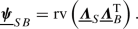(20)

Opposite to $$\underline{\varvec{{\varLambda }}}_{B}$$, which is well defined along the beam centerline, there is no obvious or unique definition for $$\underline{\varvec{{\varLambda }}}_{S}$$ in the solid domain. In Sect.  5, different definitions of the solid triad $$\underline{\varvec{{\varLambda }}}_{S}$$ are presented and investigated. However, for the derivation of the coupling equations, it is sufficient to assume the general form $$\underline{\varvec{{\varLambda }}}_{S}= \underline{\varvec{{\varLambda }}}_{S}(\underline{\varvec{{F}}})$$, i.e. formulating the solid triad as a general function of the solid deformation gradient in the current configuration.

The formulation of the constraint equations along the beam centerline brings about an advantageous property of the BTS-FULL method: the translational (18) and rotational (19) coupling constraints are completely decoupled. Therefore, the rotational coupling equations (19) can be interpreted as a direct extension to the BTS-TRANS method, which only couples the beam centerline positions to the solid as derived and thoroughly discussed in . In what follows, two different constraint enforcement strategies for the rotational coupling conditions will be presented.

### Remark 4.1

In Sect. 7, we compare the BTS-FULL method to a full 2D-3D coupling approach that enforces constraints at the 2D beam-solid interface. The governing equations, as well as the discretized coupling terms for this 2D-3D coupling scheme are stated in Appendices 2 and 3.

#### Penalty potential

We consider a quadratic space-continuous penalty potential between beam cross-section triads and solid triads defined along the beam centerline:

\begin{aligned} \varPi _{{\epsilon _\theta }}= \int _{\varGamma _{c}}{ \pi _{{\epsilon _\theta }} \,\mathrm d s}\, = \int _{\varGamma _{c}}{ \frac{1}{2} \underline{\varvec{{\psi }}}_{SB}^{\mathrm T}\underline{\varvec{{c}}}\underline{\varvec{{\psi }}}_{SB} \,\mathrm d s}\,, \end{aligned}
(21)

with the cross-section coupling potential $$\pi _{{\epsilon _\theta }}= \pi _{{\epsilon _\theta }}(s)$$ and the symmetric penalty tensor $$\underline{\varvec{{c}}}\in {\mathbb {R}}^{3\times 3}$$. Variation of the penalty potential leads to the following contribution to the weak form: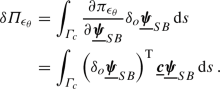(22)

Therein, $$\delta _{o}\underline{\varvec{{\psi }}}_{SB}$$ is the objective variation of the rotation vector $$\underline{\varvec{{\psi }}}_{SB}$$. Making use of (9), the variation of the total potential becomes, cf. ,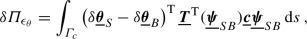(23)

where $$\delta \underline{\varvec{{\theta }}}_{S}$$ and $$\delta \underline{\varvec{{\theta }}}_{B}$$ are multiplicative variations associated with the solid and beam triad, respectively. Here, we consider penalty tensors of the form $$\underline{\varvec{{c}}}= \epsilon _{\theta }\underline{\varvec{{I}}}$$ with a scalar penalty parameter $$\epsilon _{\theta }\in {\mathbb {R}}^{+}$$ with physical unit $$\hbox {Nm/m}$$. With this definition and the identity $$\underline{\varvec{{T}}}^{\mathrm T}(\underline{\varvec{{\psi }}}) \underline{\varvec{{\psi }}}= \underline{\varvec{{\psi }}}$$ (cf. Remark 3.1) the variation of the penalty potential simplifies to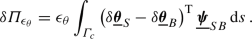(24)

It is well-known from the geometrically exact beam theory that the (multiplicative) virtual rotations $$\delta \underline{\varvec{{\theta }}}_{B}$$ are work-conjugated to the moment stress resultants. Therefore, $$\epsilon _{\theta }\underline{\varvec{{\psi }}}_{SB}$$ can be directly interpreted as the (negative) coupling moment acting on the beam cross-section.

#### Lagrange multiplier potential

Alternatively, the Lagrange multiplier method can be employed to impose the rotational coupling constraints. A Lagrange multiplier field $$\underline{\varvec{{\lambda }}}_{\theta }= \underline{\varvec{{\lambda }}}_{\theta }(s) \in {\mathbb {R}}^{3}$$ is therefore defined on the coupling curve $$\varGamma _{c}$$. For now, this field is a purely mathematical construct in the sense of generalized coupling forces associated with the coupling conditions (19). The Lagrange multiplier potential for the rotational coupling is

\begin{aligned} \varPi _{\lambda _\theta }= \int _{\varGamma _{c}}{ \underline{\varvec{{\lambda }}}_{\theta }^{\mathrm T}\underline{\varvec{{\psi }}}_{SB} \,\mathrm d s}\,. \end{aligned}
(25)

Variation of the Lagrange multiplier potential again leads to a constraint contribution to the weak form, i.e.

\begin{aligned} \delta \varPi _{\lambda _\theta }= \underbrace{\int _{\varGamma _{c}}{ \delta \underline{\varvec{{\lambda }}}_{\theta }^{\mathrm T}\underline{\varvec{{\psi }}}_{SB} \,\mathrm d s}\,}_{\delta W_{\lambda _\theta }} + \underbrace{\int _{\varGamma _{c}}{ \underline{\varvec{{\lambda }}}_{\theta }^{\mathrm T}\delta _{o}\underline{\varvec{{\psi }}}_{SB} \,\mathrm d s}\,}_{-\delta W_{C_\theta }}. \end{aligned}
(26)

Therein, $$\delta W_{\lambda _\theta }$$ and $$\delta W_{C_\theta }$$ are the variational form of the coupling constraints and the virtual work of the generalized coupling forces $$\underline{\varvec{{\lambda }}}_{\theta }$$, respectively. With (9) the virtual work of the generalized coupling forces becomes

\begin{aligned} -\delta W_{C_\theta }= \int _{\varGamma _{c}}{ (\delta \underline{\varvec{{\theta }}}_{S}- \delta \underline{\varvec{{\theta }}}_{B})^{\mathrm T}\underline{\varvec{{T}}}^{\mathrm T}(\underline{\varvec{{\psi }}}_{SB}) \underline{\varvec{{\lambda }}}_{\theta } \,\mathrm d s}\,. \end{aligned}
(27)

Since the multiplicative rotation variations $$\delta \underline{\varvec{{\theta }}}_{B}$$ are work-conjugated to the moment stress resultants of the beam, the term $$-\underline{\varvec{{T}}}^{\mathrm T}(\underline{\varvec{{\psi }}}_{SB}) \underline{\varvec{{\lambda }}}_{\theta }$$ can be interpreted as a distributed coupling moment acting along the beam centerline.

### Remark 4.2

For a vanishing relative rotation $$\underline{\varvec{{\psi }}}_{21} = \underline{\varvec{{0}}}$$, as enforced in the space-continuous problem setting according to (19), the identity $$-\underline{\varvec{{T}}}^{\mathrm T}(\underline{\varvec{{\psi }}}_{SB}) = \underline{\varvec{{I}}}$$ holds true and the rotational Lagrange multipliers exactly represent the coupling moments along the beam centerline. However, for the discretized problem this is only an approximation.

#### Objectivity of full beam-to-solid volume coupling

As indicated above, the solid triad field depends on the solid deformation gradient $$\underline{\varvec{{F}}}$$. It can easily be shown, that the presented solid triad definitions $${\hbox {STR}-\text {POL}}$$, $${\hbox {STR}-{\text {AVG}}}$$ and $${\hbox {STR}-\text {ORT}}$$, in Sect. 5 are objective with respect to an arbitrary rigid body rotation $$\underline{\varvec{{R}}}^{*}\in SO^3$$, i.e.

\begin{aligned} \underline{\varvec{{\varLambda }}}_{S}^* = \underline{\varvec{{\varLambda }}}_{S}(\underline{\varvec{{R}}}^{*}\underline{\varvec{{F}}})=\underline{\varvec{{R}}}^{*}\underline{\varvec{{\varLambda }}}_{S}(\underline{\varvec{{F}}}). \end{aligned}
(28)

The geometrically exact beam model employed in this contribution is also objective [36, 38], i.e.

\begin{aligned} \underline{\varvec{{\varLambda }}}_{B}^* = \underline{\varvec{{R}}}^{*}\underline{\varvec{{\varLambda }}}_{B}. \end{aligned}
(29)

Equations  (28) and (29) inserted into the definition of the relative beam-to-solid rotation vector according to (20) gives the rotated relative rotation vector,

\begin{aligned} \underline{\varvec{{\psi }}}_{SB}^* = {{\,\mathrm{rv}\,}}(\underline{\varvec{{R}}}^{*}\underline{\varvec{{\varLambda }}}_{S}\underline{\varvec{{\varLambda }}}_{B}^{\mathrm T}{\underline{\varvec{{R}}}^{*}}^{\mathrm T}) = \underline{\varvec{{R}}}^{*}\underline{\varvec{{\psi }}}_{SB}, \end{aligned}
(30)

where the identity $${{\,\mathrm{rv}\,}}(\underline{\varvec{{R}}}^{*}\underline{\varvec{{\varLambda }}}{\underline{\varvec{{R}}}^{*}}^{\mathrm T}) = \underline{\varvec{{R}}}^{*}{{\,\mathrm{rv}\,}}(\underline{\varvec{{\varLambda }}})$$ has been used. Thus, the rotational coupling conditions (19) in combination with the proposed solid triad definitions and the employed geometrically exact beam models are objective. As shown in , in this case also an associated penalty potential of type (21) or an associated Lagrange multiplier potential of type (25) is objective.

The previous considerations show objectivity of the proposed (space-continuous) 1D-3D coupling approaches. However, in the realm of the finite element method, cf. Sect. 6, it is important to demonstrate that objectivity is preserved also in the discrete problem setting. It is well known that the discretized deformation gradient, as required for the definition of solid triads, is objective as long as standard discretization schemes (e.g. via Lagrange polynomials) are applied to the displacement field of the solid. Also the employed beam finite element formulation based on the geometrically exact beam theory is objective, even though this topic is not trivial and the interested reader is referred to [36, 38]. Therefore, it can be concluded that the proposed 1D-3D coupling schemes are objective for the space-continuous as well as for the spatially discretized problem setting.

### Remark 4.3

Objectivity is the main reason for formulating the rotational coupling constraints (19) based on the relative rotation vector, i.e. $$\underline{\varvec{{\psi }}}_{SB}= \underline{\varvec{{0}}}$$, cf. . As alternative choice for the rotational coupling constraints the difference between the beam and solid triad rotation vectors, i.e. $$\underline{\varvec{{\psi }}}_{B}- \underline{\varvec{{\psi }}}_{S}= \underline{\varvec{{0}}}$$, could be considered. However, such coupling constraints would result in a non-objective coupling formulation .

## Definition of solid triad field

One of the main aspects of the present work is the definition of a suitable right-handed orthonormal triad field $$\underline{\varvec{{\varLambda }}}_{S}$$ in the solid, which is required for the coupling constraint (19). This is by no means a straightforward choice, and different triad definitions will lead to different properties of the resulting numerical coupling scheme. In the following, a brief motivation will be given for the concept of solid triads before different solid triad definitions will be proposed.

### Motivation of the solid triad concept

If the embedded beam is considered as a 3D body, a consistent 2D-3D coupling constraint between the 2D beam surface and the surrounding 3D solid can be formulated as

\begin{aligned} \underline{\varvec{{x}}}_B - \underline{\varvec{{x}}}_S= \underline{\varvec{{0}}}\quad \text {on} \quad \varGamma _{\text {2D-3D}}. \end{aligned}
(31)

Therein, $$\varGamma _{\text {2D-3D}}$$ is the 2D-3D coupling surface, i.e. the part of the beam surface that lies withing the solid volume. In the following, let $$\underline{\varvec{{X}}}_{S,r}$$ denote the line of material solid points that coincide with the beam centerline in the reference configuration, i.e. $$\underline{\varvec{{X}}}_{S,r}=\underline{\varvec{{r}}}_0$$. Furthermore, the orthonormal triad $$\underline{\varvec{{\varLambda }}}_{S,0}= [\underline{\varvec{{g}}}_{S1,0}, \underline{\varvec{{g}}}_{S2,0}, \underline{\varvec{{g}}}_{S3,0}]$$ shall represent material directions of the solid that coincide with the beam triad in the reference configuration according to

\begin{aligned} \underline{\varvec{{\varLambda }}}_{S,0}= \underline{\varvec{{\varLambda }}}_{B,0}. \end{aligned}
(32)

The corresponding quantities in the deformed configuration are denoted as $$\underline{\varvec{{x}}}_{S,r}$$ and $$\underline{\varvec{{\varLambda }}}_{S}$$. Let us now expand the position field in the solid as Taylor series around $$\underline{\varvec{{x}}}_{S,r}$$, i.e.

\begin{aligned} \underline{\varvec{{x}}}_S= \underline{\varvec{{x}}}_{S,r}+ \underline{\varvec{{F}}}\,\, \varDelta \underline{\varvec{{X}}} + O( \varDelta \underline{\varvec{{X}}}^2), \end{aligned}
(33)

where $$\underline{\varvec{{F}}}$$ is the deformation gradient of the solid according to (12). The 1D-3D coupling strategy underlying the proposed BTS-FULL scheme relies on the basic assumption of slender beams, i.e. $$R \ll L$$, where R is a characteristic cross-section dimension (e.g. the radius of circular cross-section s). This assumption allows to truncate the Taylor series after the linear term as long as small increments $$\varDelta \underline{\varvec{{X}}}=\alpha \underline{\varvec{{g}}}_{S2,0} + \beta \underline{\varvec{{g}}}_{S3,0}$$, with $$\alpha ,\beta \le R$$, are considered:

\begin{aligned} \underline{\varvec{{x}}}_S\approx \underline{\varvec{{x}}}_{S,r}+ \alpha \underline{\varvec{{g}}}_{S2} + \beta \underline{\varvec{{g}}}_{S3}, \end{aligned}
(34)

which results in an error of order $${\mathcal {O}}(R^2)$$. Here, the directors $${\underline{\varvec{{g}}}}_{S2}$$ and $${\underline{\varvec{{g}}}}_{S3}$$, which are not orthonormal in general, represent the push-forward of the solid directions $$\underline{\varvec{{g}}}_{S2,0}$$ and $$\underline{\varvec{{g}}}_{S3,0}$$:

\begin{aligned} \underline{\varvec{{g}}}_{Si}:=\underline{\varvec{{F}}}\,\, \underline{\varvec{{g}}}_{Si,0} \quad \text {for} \quad i=2,3. \end{aligned}
(35)

It follows from (34) and (15) that the 2D-3D coupling conditions (31) between the beam surface and the expanded solid position field are exactly fulfilled if the following 1D-3D coupling constraints are satisfied:

\begin{aligned} \underline{\varvec{{x}}}_{S,r}&=\underline{\varvec{{r}}}, \end{aligned}
(36)
\begin{aligned} \underline{\varvec{{g}}}_{S2}&=\underline{\varvec{{g}}}_{B2}, \quad \underline{\varvec{{g}}}_{S3}=\underline{\varvec{{g}}}_{B3}. \end{aligned}
(37)

Coupling constraints of the form (37) enforce that the material fibers $$\underline{\varvec{{g}}}_{S2}$$ and $$\underline{\varvec{{g}}}_{S3}$$ of the solid remain orthonormal during deformation, thus enforcing vanishing in-plane strains of the solid at the coupling point $$\underline{\varvec{{x}}}_{S,r}=\underline{\varvec{{r}}}$$. In Sect. 7, it will be demonstrated that constraints of this type lead to severe locking effects when applied to finite element discretizations that are relevant for the proposed BTS-FULL scheme, i.e. solid mesh sizes that are larger than the beam cross-section dimensions. It will be demonstrated that such locking effects can be avoided if the solid triad field is defined in a manner that only captures the purely rotational contributions to the local solid deformation at $$\underline{\varvec{{x}}}_{S,r}=\underline{\varvec{{r}}}$$ without additionally constraining the solid directors in the deformed configuration. As will be demonstrated in the next sections, the rotation tensor defined by the polar decomposition of the deformation gradient is an obvious choice for this purpose, but also alternative solid triad definitions are possible. Table 1 gives an overview of the solid triad variants proposed in the following.

All of these solid triad definitions $$\underline{\varvec{{\varLambda }}}_{S}=[\tilde{\underline{\varvec{{g}}}}_{S1}, \tilde{\underline{\varvec{{g}}}}_{S2}, \tilde{\underline{\varvec{{g}}}}_{S3}]$$ will be a function of the solid deformation gradient $$\underline{\varvec{{F}}}$$, i.e. $$\underline{\varvec{{\varLambda }}}_{S}= \underline{\varvec{{\varLambda }}}_{S}(\underline{\varvec{{F}}})$$. Moreover, all solid triad definitions will be constructed in a manner such that the associated orthonormal base vectors $$\tilde{\underline{\varvec{{g}}}}_{S2}$$ and $$\tilde{\underline{\varvec{{g}}}}_{S3}$$ represent the effective rotation of the non-orthonormal directors $${\underline{\varvec{{g}}}}_{S2}$$ and $${\underline{\varvec{{g}}}}_{S3}$$ in an average sense. Thus, it will be required that $$\tilde{\underline{\varvec{{g}}}}_{S2}$$ and $$\tilde{\underline{\varvec{{g}}}}_{S3}$$ lie within a plane defined by the normal vector

\begin{aligned} \underline{\varvec{{n}}} = \frac{{\underline{\varvec{{g}}}}_{S2} \times {\underline{\varvec{{g}}}}_{S3}}{\left\| {\underline{\varvec{{g}}}}_{S2} \times {\underline{\varvec{{g}}}}_{S3}\right\| }, \end{aligned}
(38)

in the following denoted as the $$\underline{\varvec{{n}}}$$-plane. Eventually, in the examples in Sect. 7, two desirable properties of the solid triad field for the proposed BTS-FULL method are identified:

1. (i)

The solid triad should be invariant, i.e. symmetric/ unbiased with respect to the reference in-plane beam cross-section basis vectors $$\underline{\varvec{{g}}}_{B2,0}$$ and $$\underline{\varvec{{g}}}_{B3,0}$$.

2. (ii)

The resulting BTS-FULL method should not lead to locking effects in the spatially discretized coupled problem.

These properties will be investigated for the following solid triad definitions.

### Polar decomposition of the deformation gradient ($${\hbox {STR}-\text {POL}}$$)

Based on polar decomposition, the deformation gradient of the solid problem can be split into a product $$\underline{\varvec{{F}}}= \underline{\varvec{{v}}} \underline{\varvec{{R}}}_S = \underline{\varvec{{R}}}_S \underline{\varvec{{U}}}$$ consisting of a rotation tensor $$\underline{\varvec{{R}}}_S \in SO^3$$ and a (spatial or material) positive definite symmetric tensor $$\underline{\varvec{{v}}}$$ or $$\underline{\varvec{{U}}}$$, respectively, which describes the stretch. An explicit calculation rule for the rotation tensor, e.g. based on $$\underline{\varvec{{v}}}$$, can be stated as:

\begin{aligned} \underline{\varvec{{v}}}^2&= \underline{\varvec{{F}}}\underline{\varvec{{F}}}^{\mathrm T}, \end{aligned}
(39)
\begin{aligned} \underline{\varvec{{R}}}_S&= \underline{\varvec{{v}}}^{-1} \underline{\varvec{{F}}}. \end{aligned}
(40)

As mentioned above, it is desirable that the orthonormal base vectors $$\tilde{\underline{\varvec{{g}}}}_{S2}$$ and $$\tilde{\underline{\varvec{{g}}}}_{S3}$$ of the solid triad $$\underline{\varvec{{\varLambda }}}_{S}$$ lie in a plane with normal vector $$\underline{\varvec{{n}}}$$ according to (38). It can easily be verified that the rotation tensor $$\underline{\varvec{{R}}}_S$$ associated with the total deformation gradient $$\underline{\varvec{{F}}}$$ according to (40) will in general not satisfy this requirement. Thus, a modification will be presented in the following to preserve this property.

#### Construction of $${\hbox {STR}-\text {POL}}$$ triad

Since the sought-after solid triad shall be uniquely defined already by the two in-plane directors $$\underline{\varvec{{g}}}_{S2}$$ and $$\underline{\varvec{{g}}}_{S3}$$, a modified version of the deformation gradient will be considered,

\begin{aligned} \underline{\varvec{{F}}}^{\underline{\varvec{{n}}}} = \underline{\varvec{{n}}}\otimes \underline{\varvec{{g}}}_{S1,0} + \underline{\varvec{{g}}}_{S2} \otimes \underline{\varvec{{g}}}_{S2,0} + \underline{\varvec{{g}}}_{S3} \otimes \underline{\varvec{{g}}}_{S3,0}, \end{aligned}
(41)

which consists of the projection of the total deformation gradient $$\underline{\varvec{{F}}}$$ into the $$\underline{\varvec{{n}}}$$-plane extended by the additional term $$\underline{\varvec{{n}}}\otimes \underline{\varvec{{g}}}_{S1,0}$$. This modified deformation gradient ensures that the two relevant in-plane basis vectors are correctly mapped, i.e. $$\underline{\varvec{{g}}}_{S2}=\underline{\varvec{{F}}}^{\underline{\varvec{{n}}}}\underline{\varvec{{g}}}_{S2,0}$$ and $$\underline{\varvec{{g}}}_{S3}=\underline{\varvec{{F}}}^{\underline{\varvec{{n}}}}\underline{\varvec{{g}}}_{S3,0}$$, while the third basis vector, which is not relevant for the proposed coupling procedure, is mapped onto the normal vector of the $$\underline{\varvec{{n}}}$$-plane, i.e. $$\underline{\varvec{{n}}}= \underline{\varvec{{F}}}^{\underline{\varvec{{n}}}}\underline{\varvec{{g}}}_{S1,0}$$. This specific definition of a deformation gradient allows for the following multiplicative split:

\begin{aligned} \underline{\varvec{{F}}}^{\underline{\varvec{{n}}}}= \underline{\varvec{{F}}}^{\text {2D}}\underline{\varvec{{R}}}^{\underline{\varvec{{n}}}}, \end{aligned}
(42)

where $$\underline{\varvec{{R}}}^{\underline{\varvec{{n}}}}$$ describes the (pure) rotation from the initial solid triad $$\underline{\varvec{{\varLambda }}}_{S,0}$$ onto a (still to be defined) orthonormal intermediate triad $${\bar{\underline{\varvec{{\varLambda }}}}}=[\bar{\underline{\varvec{{g}}}}_{1},\bar{\underline{\varvec{{g}}}}_{2},\bar{\underline{\varvec{{g}}}}_{3}]$$, whose base vectors $$\bar{\underline{\varvec{{g}}}}_{2}$$ and $$\bar{\underline{\varvec{{g}}}}_{3}$$ lie within the $$\underline{\varvec{{n}}}$$-plane, and $$\underline{\varvec{{F}}}^{\text {2D}}$$ represents a (quasi-2D) in-plane deformation between $$\bar{\underline{\varvec{{g}}}}_{2}$$ and $$\bar{\underline{\varvec{{g}}}}_{3}$$ and the non-orthonormal base vectors $${\underline{\varvec{{g}}}}_{2}$$ and $${\underline{\varvec{{g}}}}_{3}$$. Now, by applying the polar decomposition only to the in-plane deformation, i.e.

\begin{aligned} \underline{\varvec{{F}}}^{\text {2D}}= \underline{\varvec{{v}}}^{\text {2D}}\underline{\varvec{{R}}}_{S}^{\text {2D}}, \end{aligned}
(43)

a solid triad can be defined from the initial triad $$\underline{\varvec{{\varLambda }}}_{S,0}$$ as:

\begin{aligned} \underline{\varvec{{\varLambda }}}_{S,\text {POL}} = \underline{\varvec{{R}}}_{S}^{\text {2D}}\underline{\varvec{{R}}}^{\underline{\varvec{{n}}}}\underline{\varvec{{\varLambda }}}_{S,0}. \end{aligned}
(44)

Once an intermediate triad $${\bar{\underline{\varvec{{\varLambda }}}}}$$ is defined, the required rotation tensors $$\underline{\varvec{{R}}}_{S}^{\text {2D}}$$ and $$\underline{\varvec{{R}}}^{\underline{\varvec{{n}}}}$$ can be calculated as follows:

1. 1.

$$\underline{\varvec{{R}}}^{\underline{\varvec{{n}}}}= {\bar{\underline{\varvec{{\varLambda }}}}} \underline{\varvec{{\varLambda }}}_{S,0}^{\mathrm T}$$,

2. 2.

$$\underline{\varvec{{F}}}^{\text {2D}}= \underline{\varvec{{F}}}^{\underline{\varvec{{n}}}}(\underline{\varvec{{R}}}^{\underline{\varvec{{n}}}})^{\mathrm T}$$,

3. 3.

$$(\underline{\varvec{{v}}}^{\text {2D}})^2 = \underline{\varvec{{F}}}^{\text {2D}}(\underline{\varvec{{F}}}^{\text {2D}})^{\mathrm T}$$,

4. 4.

$$\underline{\varvec{{R}}}_{S}^{\text {2D}}= (\underline{\varvec{{v}}}^{\text {2D}})^{-1} \underline{\varvec{{F}}}^{\text {2D}}$$.

The last remaining question is the definition of the triad $${\bar{\underline{\varvec{{\varLambda }}}}}$$. It can be shown that the choice of this triad is arbitrary and does not influence the result, since a corresponding in-plane rotation offset would be automatically considered/compensated (in the sense of a superposed rigid body rotation) via the rotational part $$\underline{\varvec{{R}}}_{S}^{\text {2D}}$$ of the in-plane polar decomposition (43). For example, a simple choice is given by $$\bar{\underline{\varvec{{g}}}}_{1}\!=\underline{\varvec{{n}}}$$, $$\bar{\underline{\varvec{{g}}}}_{2}\!={\underline{\varvec{{g}}}}_{S2}/ \Vert {\underline{\varvec{{g}}}}_{S2}\Vert$$ and $$\bar{\underline{\varvec{{g}}}}_{3}\!=\underline{\varvec{{n}}} \times \bar{\underline{\varvec{{g}}}}_{2}$$, which coincides with the solid triad definition later discussed in Sect. 5.3.1.

### Remark 5.1

It can be verified that $$\underline{\varvec{{R}}}_{S}= \underline{\varvec{{R}}}_{S}^{\text {2D}}\underline{\varvec{{R}}}^{\underline{\varvec{{n}}}}$$ is fulfilled for quasi-2D deformation states, e.g. for pure torsion load cases where the beam axis remains straight during the entire deformation (see example in Sect. 7.5). In this case, the (simpler) polar decomposition of the total deformation gradient $$\underline{\varvec{{F}}}$$ according to (40) can exploited.

#### Properties of $${\hbox {STR}-\text {POL}}$$ triad

In contrast to alternative solid triad definitions that will be investigated in the following sections, the definition according to (44), referred to as $${\hbox {STR}-\text {POL}}$$ or by the subscript $$(\cdot )_{\text {POL}}$$, is not biased by an ad-hoc choice of material directors in the solid that are coupled to the beam. Instead, the rotation tensor $$\underline{\varvec{{R}}}_{S}$$ describes the rotation of material directions coinciding with the principle axes of the deformation (i.e. it maps the principle axes from the reference to the spatial configuration), which has two important implications: First, the choice of material directions that are coupled depend on the current deformation state and will in general vary in time. Second, the principle axes represent an orthonormal triad per definition, and, thus the coupling to the beam triad will not impose any constraints on the local in-plane deformation of the solid. Consequently, this solid triad variant fulfills both requirements (i) and (ii) as stated above.

Eventually, a further appealing property of the $${\hbox {STR}-\text {POL}}$$ triad shall be highlighted. Let $$\theta _0 \in [-\pi , \pi ]$$ represent the orientation of arbitrary in-plane directors in the reference configuration defined to coincide for solid and beam according to $$\underline{\varvec{{g}}}_{S,0}(\theta _0)=\underline{\varvec{{g}}}_{B,0}(\theta _0)=\cos {(\theta _0)} \, \underline{\varvec{{g}}}_{B2,0} +\sin {(\theta _0)} \, \underline{\varvec{{g}}}_{B3,0}$$. Their push-forward is given by $$\underline{\varvec{{g}}}_{S}(\theta _S(\theta _0)) = \underline{\varvec{{F}}}^{\underline{\varvec{{n}}}}\, \underline{\varvec{{g}}}_{S,0}(\theta _0)$$ for the solid and $$\underline{\varvec{{g}}}_{B}(\theta _B(\theta _0)) = \underline{\varvec{{R}}}_B \, \underline{\varvec{{g}}}_{B,0}(\theta _0)$$ for the beam, where the angles $$\theta _S \in [-\pi ,\pi ]$$ and $$\theta _B \in [-\pi ,\pi ]$$ represent the corresponding in-plane orientations in the deformed configuration (see Appendix 1 for a detailed definition). Since in-plane shear deformation is permissible for the solid but not for the beam, the orientations $$\theta _S(\theta _0)$$ and $$\theta _B(\theta _0)$$ cannot be identical for all $$\theta _0 \in [-\pi ,\pi ]$$ and arbitrary deformation states. However, as demonstrated in Appendix 1, when coupling the beam triad to the $${\hbox {STR}-\text {POL}}$$ triad according to (44), the beam directors $$\underline{\varvec{{g}}}_{B}(\theta _B(\theta _0)$$ represent the orientation of the solid directors $$\underline{\varvec{{g}}}_{S}(\theta _S(\theta _0)$$ in an average sense such that the following $$L_2$$-norm is minimized:

\begin{aligned} \int \limits _{-\pi }^{\pi } \! (\theta _S(\theta _0)-\theta _B(\theta _0))^2 \mathrm d \theta _0 \rightarrow \text {min.} \,\,\, \text {for} \,\,\, \underline{\varvec{{\varLambda }}}_{B}\!=\! \underline{\varvec{{\varLambda }}}_{S,\text {POL}}.\! \end{aligned}
(45)

In conclusion, $${\hbox {STR}-\text {POL}}$$ is an obvious choice for the solid triad with many favorable properties, e.g. it represents the average orientation of material solid directions in a $$L_2$$-optimal manner. However, it requires the calculation of the square root of a tensor, and more importantly, for latter variation and linearization procedures also the first and second derivatives of the tensor square root with respect to the solid degrees of freedom. This results in considerable computational costs, since this operation has to be performed at local Gauss point level. Therefore, alternative solid triad definitions will be proposed in the following that can be computed more efficiently, while still being able to represent global system responses with sufficient accuracy.

All solid triad variants considered in the following rely on the non-orthonormal solid directors $$\underline{\varvec{{g}}}_{S2}$$ and $$\underline{\varvec{{g}}}_{S3}$$ according to (35), their normalized counterparts

\begin{aligned} \underline{\varvec{{g}}}_{S i}':=\frac{\underline{\varvec{{g}}}_{Si}}{\left\| \underline{\varvec{{g}}}_{Si}\right\| } \quad \text {for} \quad i=2,3 \end{aligned}
(46)

and the corresponding normal vector $$\underline{\varvec{{n}}}$$ according to (38). Based on these definitions, three different variants will be exemplified in the following.

#### Fixed single solid director ($${\hbox {STR}-{\text {DIR}_{\text {2/3}}}}$$)

In the first variant, denoted as $${\hbox {STR}-{\text {DIR}_{\text {2/3}}}}$$, the orientation of one single solid director, either $$\underline{\varvec{{g}}}_{S2}'$$ or $$\underline{\varvec{{g}}}_{S3}'$$, is fixed to the solid triad, cf. Figure 3(b). The choice which solid material direction to couple is arbitrary. Therefore, two variants will be distinguished:

\begin{aligned} \underline{\varvec{{\varLambda }}}_{S,{\text {DIR}_{2}}}&= [\begin{array}{l} \underline{\varvec{{n}}}, \underline{\varvec{{g}}}_{S2}', \underline{\varvec{{n}}} \times \underline{\varvec{{g}}}_{S2}'\end{array} ] \end{aligned}
(47)
\begin{aligned} \underline{\varvec{{\varLambda }}}_{S,{\text {DIR}_{3}}}&= [\begin{array}{l} \underline{\varvec{{n}}}, \underline{\varvec{{g}}}_{S3}' \times \underline{\varvec{{n}}}, \underline{\varvec{{g}}}_{S3}' \end{array}] , \end{aligned}
(48)

Since the variant $${\hbox {STR}-{\text {DIR}_{\text {2/3}}}}$$ does not fulfill the requirement (i) as stated above, it will only be considered for comparison reasons in the 2D verification examples in Sect. 7.

#### Fixed average solid director ($${\hbox {STR}-{\text {AVG}}}$$)

In order to solve this problem, i.e. to define a solid triad that is symmetric with respect to the base vectors $$\underline{\varvec{{g}}}_2'$$ and $$\underline{\varvec{{g}}}_3'$$, an alternative variant denoted as $${\hbox {STR}-{\text {AVG}}}$$ is proposed, which relies on the average of the directors $$\underline{\varvec{{g}}}_2'$$ and $$\underline{\varvec{{g}}}_3'$$, cf. Fig. 3(c):

\begin{aligned} \underline{\varvec{{g}}}_{S,{\text {AVG}}}= \frac{ \underline{\varvec{{g}}}_{S2}' + \underline{\varvec{{g}}}_{S3}' }{\left\| \underline{\varvec{{g}}}_{S2}' + \underline{\varvec{{g}}}_{S3}' \right\| }. \end{aligned}
(49)

With this average vector the solid triad can be constructed as: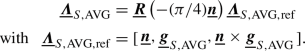(50)

The rotation tensor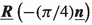in (50) represents a “back-rotation” of the constructed reference triad $$\underline{\varvec{{\varLambda }}}_{S,{\text {AVG}},\text {ref}}$$ by an angle of $$-\pi /4$$ to ensure that the resulting solid triad aligns with the beam triad in the reference configuration according to (32). In Sect. 7, it will be shown numerically that the variant $${\hbox {STR}-{\text {AVG}}}$$, similar to the variant $${\hbox {STR}-\text {POL}}$$, fulfills both requirements (i) and (ii) stated above.

### Remark 5.2

Theoretically, an additive director averaging procedure such as (49) can result in a singularity if the underlying vectors are anti-parallel, i.e. $$\underline{\varvec{{g}}}_{S2}' =- \underline{\varvec{{g}}}_{S3}'$$. However, since the associated material directors are orthogonal in the reference configuration, i.e. $$\underline{\varvec{{g}}}_{S2,0}^{\mathrm T}\underline{\varvec{{g}}}_{S3,0}=0$$, and shear angles smaller than $$\pi /2$$ can be assumed, this singularity will not be relevant for practical applications.

#### Fixed orthogonal solid material directions ($${\hbox {STR}-\text {ORT}}$$)

In the last considered solid triad definition, both material directors $$\underline{\varvec{{g}}}_{S2}'$$ and $$\underline{\varvec{{g}}}_{S3}'$$ are coupled to the solid triad simultaneously. This variant enforces that the directors $$\underline{\varvec{{g}}}_{S2}'$$ and $$\underline{\varvec{{g}}}_{S3}'$$ remain orthogonal to each other, and thus it is denoted as $${\hbox {STR}-\text {ORT}}$$, indicated by a subscript $$(\cdot )_{\text {ORT}}$$. The $${\hbox {STR}-\text {ORT}}$$ variant is realized by applying the rotational coupling constraints (19) twice, once with $$\underline{\varvec{{\varLambda }}}_{S,{\text {DIR}_{2}}}$$ according to (47) and once with $$\underline{\varvec{{\varLambda }}}_{S,{\text {DIR}_{3}}}$$ according to (48).

Opposed to the other triad definitions in this section, this version additionally imposes a constraint on the solid displacement field by enforcing all shear strain components to vanish at the coupling point. In Sect. 7, it will be demonstrated that this over-constrained solid triad definition can lead to severe shear locking effects, i.e. requirement (ii) from Sect. 5.1 is not satisfied. Thus, also this variant will only be considered for comparison reasons in the 2D verification examples in Sect. 7.

### Variation of the solid rotation vector

In the coupling contributions to the weak form (24) and (26) the multiplicative rotation vector variation $$\delta \underline{\varvec{{\theta }}}_{S}$$ (spin vector) of a solid rotation vector $$\underline{\varvec{{\psi }}}_{S}$$ arises. The spin vector is work-conjugated with the coupling moments, i.e. it is required to calculate the virtual work of a moment acting on the solid in a variationally consistent manner. In contrast to the beam spin vector $$\delta \underline{\varvec{{\theta }}}_{B}$$, which represents the multiplicative variation of primal degrees of freedom in the finite element discretization of the geometrically exact Simo–Reissner beam theory and is discretized directly, no such counterpart exists for the solid field. Therefore, it is assumed that the solid spin vector can be stated as a function of a set of generalized solid degrees of freedom $$\varvec{{q}}$$ (which will later be identified as nodal position vectors in the context of a finite element discretization) and their variations $$\delta \varvec{{q}}$$. The additive variation of the solid rotation vector $$\underline{\varvec{{\psi }}}_{S}(\varvec{{q}})$$ then reads

\begin{aligned} \delta \underline{\varvec{{\psi }}}_{S}= \frac{\partial \underline{\varvec{{\psi }}}_{S}(\varvec{{q}})}{\partial \varvec{{q}}} \delta \varvec{{q}}. \end{aligned}
(51)

The multiplicative and additive variations are related via (7), which gives the spin vector associated with the solid triad as a function of the generalized solid degrees of freedom: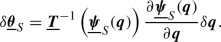(52)

### Remark 5.3

Alternatively, the solid spin vector can be expressed by the variations of the corresponding solid triad basis vectors $$\underline{\varvec{{g}}}_{Si}$$ and their variations $$\delta \underline{\varvec{{g}}}_{Si}$$, cf. [36, 38]: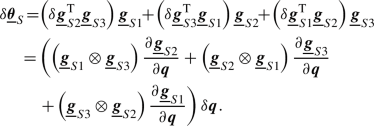This formulation for the solid spin vector is equivalent to the one in (52), but only contains the solid triad basis vectors and their variations. Therefore, this definition of the solid spin vector is better suited for solid triads constructed via their basis vector. Especially in the implementation of the finite element formulation, it is advantageous to avoid the computation and inversion of the transformation matrix in (52). Nonetheless, in the remainder of this contribution, the solid spin vector as defined in (52) is used to improve readability of the equations.

## Spatial discretization

In this work, spatial discretization of the beam, solid and coupling problem will exclusively be based on the finite element method. In the following, a subscript $$(\cdot )_h$$ refers to an interpolated field quantity, superscripts $${(e)}$$ and $${(f)}$$ indicate that the quantity is defined for a solid element $$e$$ and a beam element $$f$$, respectively. Accordingly, $${(e,f)}$$ refers to coupling terms between the solid element $$e$$ and beam element $$f$$. The global element count is made up of $$n_{\text {el},S}$$ solid finite elements and $$n_{\text {el},B}$$ beam finite elements.

### Solid and beam problem

For the solid domain an isoparametric finite element approach is used to interpolate position, displacement and virtual displacement field within each solid element $$\varOmega _{S,h}^{(e)}$$: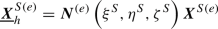(53)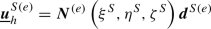(54)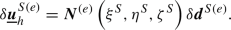(55)

Therein, $$\varvec{{N}}^{(e)}\in {\mathbb {R}}^{3\times n_{\text {dof}}^{(e)}}$$ is the element shape function matrix, which depends on the solid element parameter coordinates $$\xi ^S, \eta ^S, \zeta ^S\in {\mathbb {R}}^{}$$. Furthermore, $${\varvec{{X}}^{S{(e)}}}\in {\mathbb {R}}^{n_{\text {dof}}^{(e)}}$$, $${\varvec{{d}}^{S{(e)}}}\in {\mathbb {R}}^{n_{\text {dof}}^{(e)}}$$ and $${\delta {\varvec{{d}}^{S{(e)}}}}\in {\mathbb {R}}^{n_{\text {dof}}^{(e)}}$$ are the element reference position vector, element displacement vector and element virtual displacement vector, respectively. Each solid element has $$n_{\text {dof}}^{(e)}$$ degrees of freedom.

The beam finite elements used in this work are based on the Simo–Reissner formulation presented in [35, 38]. Figure 4. illustrates the degrees of freedom for a single beam finite element. The beam centerline interpolation is $$C^{1}$$-continuous based on third-order Hermite polynomials with two centerline nodes per element. Each node for the centerline interpolation has 6 degrees of freedom: 3 for the nodal position $${\hat{\underline{\varvec{{r}}}}}^{(f)}_i$$ and 3 for the centerline tangent $$\hat{\underline{\varvec{{t}}}}^{(f)}_i$$ at the node, thus resulting in a total of 12 element degrees of freedom describing the beam centerline position. The interpolated position of the beam centerline is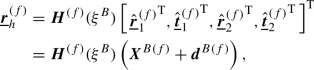(56)

with the beam position shape function matrix $$\varvec{{H}}^{(f)}\in {\mathbb {R}}^{3\times 12}$$, the beam centerline reference position vector $$\varvec{{X}}^{B{(f)}}\in {\mathbb {R}}^{12}$$ and the beam centerline displacement vector $$\varvec{{d}}^{B{(f)}}\in {\mathbb {R}}^{12}$$. Furthermore, $$\xi ^B\in {\mathbb {R}}^{}$$ is the parameter coordinate along the beam centerline.

A triad interpolation scheme based on three element nodal rotation vectors $${\hat{\underline{\varvec{{\psi }}}}}^{(f)}_1$$, $${\hat{\underline{\varvec{{\psi }}}}}^{(f)}_2$$ and $${\hat{\underline{\varvec{{\psi }}}}}^{(f)}_3$$ is utilized . The third node is placed in the middle of the element and carries no translational degrees of freedom, only rotational ones. The three local nodal rotation vectors serve as primal degrees of freedom for the interpolated rotation field along the beam centerline. Each local rotation vector has 3 degrees of freedom, thus resulting in a total of 9 rotational degrees of freedom per beam finite element. The interpolation of the beam cross-section triad along the beam centerline is a non-trivial task and requires an orthonormal interpolation scheme for the interpolated triad $$\underline{\varvec{{\varLambda }}}_{B,h}^{(f)}(\xi ^B)$$ to guarantee that the interpolated triad field is still a member of the rotational group $$SO^3$$. Furthermore, objectivity of the discrete beam deformation measures has to be preserved by the interpolation, which is a challenging task if rotational degrees of freedom are involved. In this contribution we will refer to the interpolated triad field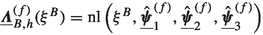as an abstract nonlinear function of the beam parameter coordinate and the nodal rotation vectors. The corresponding interpolated field of multiplicative rotation vector increments $$\varDelta \underline{\varvec{{\theta }}}_{B,h}^{(f)}(\xi ^B)$$ has been consistently derived in  and reads:

\begin{aligned} \varDelta \underline{\varvec{{\theta }}}_{B,h}^{(f)}= \sum _{i=1}^{3} \tilde{\underline{\varvec{{I}}}}^{(f)}_i (\xi ^B) \varDelta \hat{\underline{\varvec{{\theta }}}}^{(f)}_i = \tilde{\varvec{{I}}}^{(f)}(\xi ^B) \varDelta \hat{\varvec{\theta }}_{B}^{(f)}. \end{aligned}
(57)

Therein, $$\tilde{\underline{\varvec{{I}}}}^{(f)}_i \in {\mathbb {R}}^{3\times 3}$$ are generalized shape function matrices for the multiplicative nodal rotation increments $$\varDelta \hat{\underline{\varvec{{\theta }}}}^{(f)}_i$$, and $$\tilde{\varvec{{I}}}^{(f)}\in {\mathbb {R}}^{3 \times 9}$$ and $$\varDelta \hat{\varvec{\theta }}_{B}^{(f)} \in {\mathbb {R}}^{9}$$ are the corresponding element-wise assembled quantities. It should be pointed out that $$\tilde{\underline{\varvec{{I}}}}^{(f)}_i$$ are nonlinear functions of the beam parameter coordinate and the nodal rotation vectors of the beam element, i.e. these rotational shape functions are deformation-dependent. To avoid this nonlinearity in the discretized spin vector, i.e. the virtual rotation field $${\delta \underline{\varvec{{\theta }}}_{B,h}^{(f)}}$$, which would require additional linearization contributions to calculate a consistent tangent, the beam finite elements employed in this work follow a Petrov–Galerkin discretization approach. Therein, standard Lagrange shape functions are used to interpolate the discretized nodal spin vectors:

\begin{aligned} {\delta \underline{\varvec{{\theta }}}_{B,h}^{(f)}}= \sum _{i=1}^{3} L^{(f)}_i (\xi ^B) \delta \hat{\underline{\varvec{{\theta }}}}^{(f)}_i = {\varvec{{L}}^{(f)}}(\xi ^B) {\delta \hat{\varvec{{\theta }}}_B^{(f)}}. \end{aligned}
(58)

Here, $$L^{(f)}_i \in {\mathbb {R}}^{}$$ are standard second-order Lagrange polynomials, and $$\delta \hat{\underline{\varvec{{\theta }}}}^{(f)}_i$$ are the nodal spin vectors. Again, this equation can be assembled element-wise, thus resulting in the shape function matrix $${\varvec{{L}}^{(f)}}\in {\mathbb {R}}^{3 \times 9}$$ and the element spin vector $${\delta \hat{\varvec{{\theta }}}_B^{(f)}}\in {\mathbb {R}}^{9}$$.

In what follows, all coupling terms are evaluated on the beam centerline. This requires the projection of points along the beam centerline parameter space into the solid element parameter space, which in turn is achieved by solving the set of nonlinear equations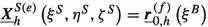, for a given $$\xi ^B$$. To improve readability, the superscripts indicating the beam and solid elements will be omitted from now on. They will however be stated in the virtual work contributions and the integration domains in order to clearly indicate pair-wise values. Additionally, any dependency on element parameter coordinates will not be stated explicitly.

### Remark 6.1

While the $$C^1$$-continuous centerline representation of the employed beam elements  is not mandatory for the considered beam-to-solid volume coupling problem, it offers significant advantages in problems additionally involving beam-to-beam  or beam-to-solid contact interaction, which will be addressed in our future research.

### Gauss point-to-segment coupling of cross-section rotations

Evaluating the variation of the total coupling potential (24) based on the discretized solid position field and beam cross-section rotation field as presented in the last section yields the discrete variation of the coupling potential: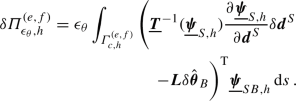(59)

Therein, $$\varGamma _{c,h}^{{(e,f)}}= \varOmega _{B,h}^{(f)}\cap \varOmega _{S,h}^{(e)}$$ is the discretized coupling domain between beam element $${(f)}$$ and solid element $${(e)}$$. The integral in (59) is evaluated numerically via a Gauss–Legendre quadrature, resulting in a Gauss point-to-segment (GPTS) coupling scheme. From a mechanical point of view this can be interpreted a weighted enforcement of the rotational constraints at each integration point along the beam, i.e. a Gauss point-to-segment type coupling: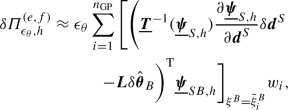(60)

where $$n_{\text {GP}}$$ is the number of Gauss–Legendre points, $${\tilde{\xi }}^B_{i}$$ is the beam element parameter coordinate for Gauss–Legendre point $$i$$ with the corresponding weight $$w_i$$. Again, in order to improve the readability of the remaining equations in this subsection, the explicit indication of the evaluation at the Gauss–Legendre points will be omitted in the following. The previous equation can now be stated in matrix form as

\begin{aligned} \begin{aligned} \delta \varPi _{{\epsilon _\theta },h}^{(e,f)}&\approx [\begin{array}{ll}\delta \hat{\varvec{{\theta }}}_B^{\mathrm T}&{\delta {{\varvec{{d}}^S}}}^{\mathrm T}\end{array}] \sum _{i=1}^{n_{\text {GP}}}{ w_{i}\left[ \begin{array}{l}\varvec{{f}}_{c,\text {GP}}^{B}\\ \varvec{{f}}_{c,\text {GP}}^{S}\end{array}\right] } \\&= \left[ \begin{array}{ll}\delta \hat{\varvec{{\theta }}}_B^{\mathrm T}&{\delta {{\varvec{{d}}^S}}}^{\mathrm T}\end{array}\right] \left[ \begin{array}{l}\varvec{{r}}_{c,\text {GP}}^B\\ \varvec{{r}}_{c,\text {GP}}^S\end{array}\right] . \end{aligned} \end{aligned}
(61)

Therein, the abbreviations $$\varvec{{f}}_{c,\text {GP}}^{B}\in {\mathbb {R}}^{9}$$ and $$\varvec{{f}}_{c,\text {GP}}^{S}\in {\mathbb {R}}^{n_{\text {dof}}^{(e)}}$$ for the generalized Gauss point coupling forces on the rotational beam degrees of freedom and the generalized solid element degrees of freedom, respectively, have been introduced: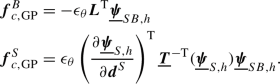(62)

Furthermore, $$\varvec{{r}}_{c,\text {GP}}^B\in {\mathbb {R}}^{9}$$ and $$\varvec{{r}}_{c,\text {GP}}^S\in {\mathbb {R}}^{n_{\text {dof}}^{(e)}}$$ are the beam and solid coupling residual vectors. Employing a Newton–Raphson algorithm to solve the global system of nonlinear equations, a linearization of the residual vectors with respect to the element degrees of freedom is required, which reads:

\begin{aligned} \left[ \begin{array}{l}\varDelta \varvec{{r}}_{c,\text {GP}}^B\\ \varDelta \varvec{{r}}_{c,\text {GP}}^S\end{array}\right] = \sum _{i=1}^{n_{\text {GP}}}{ w_{i}\left[ \begin{array}{ll} \frac{\partial \varvec{{f}}_{c,\text {GP}}^{B}}{\partial \underline{\varvec{{\psi }}}_{B,h}} \underline{\varvec{{T}}}(\underline{\varvec{{\psi }}}_{B,h}) \tilde{\varvec{{I}}}&{} \frac{\partial \varvec{{f}}_{c,\text {GP}}^{B}}{\partial {{\varvec{{d}}^S}}} \\ \frac{\partial \varvec{{f}}_{c,\text {GP}}^{S}}{\partial \underline{\varvec{{\psi }}}_{B,h}} \underline{\varvec{{T}}}(\underline{\varvec{{\psi }}}_{B,h}) \tilde{\varvec{{I}}}&{} \frac{\partial \varvec{{f}}_{c,\text {GP}}^{S}}{\partial {{\varvec{{d}}^S}}} \end{array}\right] \left[ \begin{array}{l}\varDelta \hat{\varvec{{\theta }}}_B\\ {\varDelta {{\varvec{{d}}^S}}}\end{array}\right] }.\nonumber \\ \end{aligned}
(63)

Therein, the transformation matrix $$\underline{\varvec{{T}}}(\underline{\varvec{{\psi }}}_{B,h})$$ appears, since the linearization is performed with respect to the multiplicative rotation increments $$\varDelta \underline{\varvec{{\theta }}}_{B,h}$$. Furthermore, the generalized shape function matrix $$\tilde{\varvec{{I}}}$$ follows from the interpolation of the multiplicative rotation increments, cf. (57). The previously derived matrices and vectors are all defined on beam-to-solid element pair level. Since no additional degrees of freedom are introduced, the pair-wise contributions can simply be assembled and added to the global linear system of equations. The Gauss point-to-segment coupling approach is presented here to illustrate how the rotational coupling conditions can be enforced in a point-wise manner. However, in  it has been shown that a Gauss point-to-segment coupling approach leads to spurious contact locking for embedded one-dimensional beams in three-dimensional solid volumes. Therefore, this approach will not be investigated further in the remainder of this contribution, but a mortar-type coupling is proposed instead.

### Mortar-type coupling of cross-section rotations

Employing a mortar-type coupling approach, the rotational Lagrange multiplier field $$\underline{\varvec{{\lambda }}}_{\theta }$$ introduced in Sect. 4.3.2 is also approximated with a finite element interpolation, cf. [7, 46, 71]. The rotational Lagrange multiplier field is defined along the beam centerline and accordingly its finite element approximation is defined along the beam finite element and reads as follows:

\begin{aligned} {\underline{\varvec{{\lambda }}}_{\theta ,h}^{{(f)}}}= \sum _{i=1}^{n_{\lambda }^{(f)}} \varPhi ^{(f)}_i(\xi ^B) \varvec{{\lambda }}^{(f)}_{\theta ,i}= {\varvec{{\varPhi }}^{(f)}}(\xi ^B) {\varvec{{\lambda }}_{\theta }^{(f)}}, \end{aligned}
(64)

where $$n_{\lambda }^{(f)}$$ is the number of Lagrange multiplier nodes on beam element $${(f)}$$, $$\varPhi ^{(f)}_i$$ is the shape function for the local node i and $$\varvec{{\lambda }}^{(f)}_{\theta ,i}\in {\mathbb {R}}^{3}$$ is the rotational Lagrange multiplier at node i. Furthermore, $${\varvec{{\varPhi }}^{(f)}}\in {\mathbb {R}}^{3 \times 3 n_{\lambda }^{(f)}}$$ is the element-wise assembled Lagrange multiplier shape function matrix for a beam element and $${\varvec{{\lambda }}_{\theta }^{(f)}}\in {\mathbb {R}}^{3 n_{\lambda }^{(f)}}$$ is the vector with all corresponding discrete rotational Lagrange multiplier values per beam element. As indicated by the dependency on beam parameter coordinate $$\xi ^B$$, the Lagrange multiplier field is defined along the beam centerline. However, there is no requirement that the Lagrange multiplier shape functions are identical to the beam centerline shape functions, or even that the number of beam nodes matches the number of Lagrange multiplier nodes. A more thorough discussion on the choice of Lagrange multiplier shape functions is given at the end of this section.

When inserting the finite element interpolations, the discretized variation of the coupling constraints (26) reads

\begin{aligned} \delta W_{\lambda _\theta ,h}^{(e,f)}= \delta \varvec{{\lambda }}_{\theta }^{\mathrm T}\int _{\varGamma _{c,h}^{{(e,f)}}}{ \underbrace{ \varvec{{\varPhi }}^{\mathrm T}\underline{\varvec{{\psi }}}_{SB,h}}_{\varvec{{g}}_{c,\lambda _\theta }} \,\mathrm d s}\, = \delta \varvec{{\lambda }}_{\theta }^{\mathrm T}\varvec{{r}}_{c,\lambda _\theta }. \end{aligned}
(65)

Therein, the abbreviations $$\varvec{{g}}_{c,\lambda _\theta }\in {\mathbb {R}}^{n_{\text {dof},\lambda }^{(f)}}$$ and $$\varvec{{r}}_{c,\lambda _\theta }\in {\mathbb {R}}^{n_{\text {dof},\lambda }^{(f)}}$$ represent the integrand of the pair constraint equations and the residual of the pair constraints equations, respectively. The discretized virtual work of the coupling forces (27) reads

\begin{aligned} \begin{aligned} -\delta W_{C_\theta ,h}^{(e,f)}&= \left[ \begin{array}{ll}\delta \hat{\varvec{{\theta }}}_B^{\mathrm T}&{\delta {{\varvec{{d}}^S}}}^{\mathrm T}\end{array}\right] \left[ \begin{array}{l}\int _{\varGamma _{c,h}^{{(e,f)}}}{ \varvec{{f}}_{c,\lambda _\theta }^{B} \,\mathrm d s}\, \\ \int _{\varGamma _{c,h}^{{(e,f)}}}{ \varvec{{f}}_{c,\lambda _\theta }^{S} \,\mathrm d s}\,\end{array}\right] \\&=\left[ \begin{array}{ll}\delta \hat{\varvec{{\theta }}}_B^{\mathrm T}&{\delta {{\varvec{{d}}^S}}}^{\mathrm T}\end{array}\right] \left[ \begin{array}{l}\varvec{{r}}_{c,\lambda _\theta }^B\\ \varvec{{r}}_{c,\lambda _\theta }^S\end{array}\right] . \end{aligned} \end{aligned}
(66)

Therein, the abbreviations $$\varvec{{f}}_{c,\lambda _\theta }^{B}\in {\mathbb {R}}^{9}$$ and $$\varvec{{f}}_{c,\lambda _\theta }^{S}\in {\mathbb {R}}^{n_{\text {dof}}^{(e)}}$$ represent the integrand of the beam and solid element coupling forces, i.e.

\begin{aligned} \begin{aligned} \varvec{{f}}_{c,\lambda _\theta }^{B}&= \varvec{{L}}^{\mathrm T}\underline{\varvec{{T}}}^{\mathrm T}(\underline{\varvec{{\psi }}}_{SB,h}) \varvec{{\varPhi }}\varvec{{\lambda }}_{\theta }, \\ \varvec{{f}}_{c,\lambda _\theta }^{S}&= \frac{\partial \underline{\varvec{{\psi }}}_{S,h}}{\partial {{\varvec{{d}}^S}}}^{\mathrm T}\underline{\varvec{{T}}}^{-\mathrm T}(\underline{\varvec{{\psi }}}_{S,h}) \underline{\varvec{{T}}}^{\mathrm T}(\underline{\varvec{{\psi }}}_{SB,h}) \varvec{{\varPhi }}\varvec{{\lambda }}_{\theta }. \end{aligned} \end{aligned}
(67)

Furthermore, $$\varvec{{r}}_{c,\lambda _\theta }^B\in {\mathbb {R}}^{9}$$ and $$\varvec{{r}}_{c,\lambda _\theta }^S\in {\mathbb {R}}^{n_{\text {dof}}^{(e)}}$$ are the beam and solid coupling residual vectors, respectively. Again, a linearization of the residual contributions with respect to the discrete beam-to-solid pair degrees of freedom is required for the Newton–Raphson algorithm. The linearization is:

\begin{aligned} \left[ \begin{array}{l}\varDelta \varvec{{r}}_{c,\lambda _\theta }^B\\ \varDelta \varvec{{r}}_{c,\lambda _\theta }^S\\ \varDelta \varvec{{r}}_{c,\lambda _\theta }\end{array}\right] = \left[ \begin{array}{lll} \varvec{{q}}_{SS}&{} \varvec{{q}}_{SB}&{} \varvec{{q}}_{S\lambda _\theta }\\ \varvec{{q}}_{BS}&{} \varvec{{q}}_{BB}&{} \varvec{{q}}_{B\lambda _\theta }\\ \varvec{{q}}_{\lambda _\theta S}&{} \varvec{{q}}_{\lambda _\theta B}&{} \varvec{{0}}\end{array}\right] \left[ \begin{array}{l}\varDelta \hat{\varvec{{\theta }}}_B\\ {\varDelta {{\varvec{{d}}^S}}}\\ \varvec{{\lambda }}_{\theta }\end{array}\right] . \end{aligned}
(68)

Therein, the abbreviations $$\varvec{{q}}_{(\cdot )}$$ for the stiffness matrices of the pair-wise coupling terms have been introduced, i.e.

\begin{aligned} \begin{aligned}&\left[ \begin{array}{lll} \varvec{{q}}_{SS}&{} \varvec{{q}}_{SB}&{} \varvec{{q}}_{S\lambda _\theta }\\ \varvec{{q}}_{BS}&{} \varvec{{q}}_{BB}&{} \varvec{{q}}_{B\lambda _\theta }\\ \varvec{{q}}_{\lambda _\theta S}&{} \varvec{{q}}_{\lambda _\theta B}&{} \varvec{{0}}\end{array}\right] \\&\qquad = \int _{\varGamma _{c,h}^{{(e,f)}}}{ \left[ \begin{array}{lll} \frac{\partial \varvec{{f}}_{c,\lambda _\theta }^{B}}{\partial \underline{\varvec{{\psi }}}_{B,h}} \underline{\varvec{{T}}}(\underline{\varvec{{\psi }}}_{B,h}) \tilde{\varvec{{I}}}&{} \frac{\partial \varvec{{f}}_{c,\lambda _\theta }^{B}}{\partial {{\varvec{{d}}^S}}} &{} \frac{\partial \varvec{{f}}_{c,\lambda _\theta }^{B}}{\partial \varvec{{\lambda }}_{\theta }} \\ \frac{\partial \varvec{{f}}_{c,\lambda _\theta }^{S}}{\partial \underline{\varvec{{\psi }}}_{B,h}} \underline{\varvec{{T}}}(\underline{\varvec{{\psi }}}_{B,h}) \tilde{\varvec{{I}}}&{} \frac{\partial \varvec{{f}}_{c,\lambda _\theta }^{S}}{\partial {{\varvec{{d}}^S}}} &{} \frac{\partial \varvec{{f}}_{c,\lambda _\theta }^{S}}{\partial \varvec{{\lambda }}_{\theta }} \\ \frac{\partial \varvec{{g}}_{c,\lambda _\theta }}{\partial \underline{\varvec{{\psi }}}_{B,h}} \underline{\varvec{{T}}}(\underline{\varvec{{\psi }}}_{B,h}) \tilde{\varvec{{I}}}&{} \frac{\partial \varvec{{g}}_{c,\lambda _\theta }}{\partial {{\varvec{{d}}^S}}} &{} \varvec{{0}}\end{array}\right] \,\mathrm d s}\,. \end{aligned} \end{aligned}
(69)

As in the GPTS case, the previously derived vectors and matrices are all defined on beam-to-solid element pair level. However, in this case additional unknowns have been introduced, i.e. the rotational Lagrange multipliers $$\varvec{{\lambda }}_{\theta }$$ on pair level. In practice, all derivatives explicitly stated in (66) and (68) are evaluated using forward automatic differentiation (FAD), cf. , using the Sacado software package , which is part of the Trilinos project .

At this point it should be pointed out that all coupling integrals are evaluated numerically using so-called segment-based integration, cf. [18, 63]. Therein, the beam finite element parameter space is divided into subsegments at points where the beam crosses a solid finite element face. Each subsegment is subsequently integrated using a Gauss–Legendre quadrature with a fixed number of integration points. This leads to a highly accurate numerical integration procedure and allows for the resulting finite element coupling method to pass classical patch tests in surface-to-surface problems as well as constant stress transfer tests in beam-to-solid problems, cf. [18, 63].

The choice of proper Lagrange multiplier basis functions is important for the mathematical properties of the resulting finite element discretization. The Lagrange multiplier shape functions must fulfill an $$\mathrm {inf}\text {-}\mathrm {sup}$$ condition to guarantee stability of the mixed finite element method . This is a well-studied topic in the context of classical surface-to-surface mesh tying or contact. However, as pointed out in , beam-to-solid coupling problems diverge from the standard surface-to-surface case in some aspects. First, the discretization along the beam centerline with Hermite polynomials is unusual compared to standard (i.e. Lagrange polynomial-based) finite element discretizations. Also, the 1D-3D coupling can be classified as a mixed-dimensional embedded mesh problem, since there is no explicit curve in the solid domain to match the beam centerline, which can lead to stability issues . Additionally, in this contribution we deal with rotational coupling, which also differs from the standard surface-to-surface case. A deep mathematical analysis of these properties is beyond the scope of the present contribution. However, we will build upon the extensive studies and findings from , where it has been shown that a linear interpolation of the Lagrange multipliers combined with a penalty regularization leads to a stable finite element formulation of the coupled problem. Instabilities might only occur if the beam finite elements become shorter than the solid finite elements. However, this is not a mesh size relation that is within the envisioned applications for the BTS-FULL method. Alternative approaches to avoid the mentioned instabilities are available in the literature, such as Nitsche’s method [15, 23, 58], or discontinuous Galerkin formulations [23, 57]. Another appealing approach is the so-called vital vertex method introduced in , where discrete Lagrange multipliers are inserted at intersection points between the coupled meshes, i.e. the intersections between a beam finite element centerline and the solid elements in the presented beam-to-solid case.

### Beam-to-solid volume coupling (BTS-TRANS)

In Sects. 6.2 and 6.3, GPTS and mortar-type coupling discretizations for the rotational coupling between the beam cross-section and the solid volume (19) have been presented. The translational coupling of the beam centerline (18), however, is entirely based on the mortar-type beam-to-solid volume coupling (BTS-TRANS) method previously introduced in . The resulting linearized system of equations for the centerline translation coupling with saddle point structure reads:

\begin{aligned} \left[ \begin{array}{llll} \varvec{{K}}^S&{} \varvec{{0}}&{} \varvec{{0}}&{} -\varvec{{M}}^{\mathrm T}\\ \varvec{{0}}&{} \varvec{{K}}^B_{rr}&{} \varvec{{K}}^B_{r\theta }&{} \varvec{{D}}^{\mathrm T}\\ \varvec{{0}}&{} \varvec{{K}}^B_{\theta r}&{} \varvec{{K}}^B_{\theta \theta }&{} \varvec{{0}}\\ -\varvec{{M}}&{} \varvec{{D}}&{} \varvec{{0}}&{} \varvec{{0}}\end{array}\right] \left[ \begin{array}{l}\varDelta {{\varvec{{D}}^S}}\\ \varDelta \varvec{{D}}^B_r\\ \varDelta \varvec{{D}}^B_{\theta }\\ \varvec{{\varLambda }}_{r}\end{array}\right] = \begin{bmatrix} -\varvec{{R}}^S\\ -\varvec{{R}}^B_{r}\\ -\varvec{{R}}^B_{\theta }\\ \varvec{{M}}{{\varvec{{D}}^S}}- \varvec{{D}}\varvec{{D}}^B_r\end{bmatrix}.\nonumber \\ \end{aligned}
(70)

Therein, $$\varvec{{K}}^S$$ is the solid tangent stiffness matrix, $${{\varvec{{D}}^S}}$$ and $$\varDelta {{\varvec{{D}}^S}}$$ are the global solid displacement vector and its increment, respectively, and $$\varvec{{R}}^S$$ is the residual of the solid degrees of freedom. In (70), the beam terms are split into centerline and rotation contributions indicated by $$(\cdot )_{r}$$ and $$(\cdot )_{\theta }$$, respectively. At this point it should be noted that due to the employed Petrov–Galerkin method the beam stiffness matrices are non-symmetric, as will be the case for the rotational coupling contributions to the global stiffness matrix. Furthermore, $$\varvec{{D}}^B_r$$ and $$\varDelta \varvec{{D}}^B_r$$ are the global beam centerline displacement vector and its increment, and $$\varDelta \varvec{{D}}^B_{\theta }$$ represents the global vector collecting the multiplicative rotation increments associated with the nodal triads of the beam finite elements. The update of the rotation state has to be preformed according to . The BTS-TRANS coupling is represented by the discrete mortar matrices $$\varvec{{D}}$$ and $$\varvec{{M}}$$ and the centerline Lagrange multipliers $$\varvec{{\varLambda }}_{r}$$. The structure of the global stiffness matrix in (70) illustrates that only the beam centerline degrees of freedom (and not the rotational degrees of freedom) are coupled to the solid degrees of freedom in the previously proposed BTS-TRANS coupling scheme.

### Combined mortar-type coupling of translations and rotations (BTS-FULL)

The global system of equations for the mortar-type BTS-FULL method is the combination of the mortar-type coupling for the centerline positions BTS-TRANS (70) and the mortar-type coupling of the beam cross-section rotations (68). The resulting global system of equations becomes:

\begin{aligned}&\!\!\!\left[ \begin{array}{llllll} \varvec{{K}}^S+ \varvec{{Q}}_{SS}&{} \varvec{{0}}&{} \varvec{{Q}}_{SB}&{} -\varvec{{M}}^{\mathrm T}&{} \varvec{{Q}}_{S\lambda _\theta }\\ \varvec{{0}}&{} \varvec{{K}}^B_{rr}&{} \varvec{{K}}^B_{r\theta }&{} \varvec{{D}}^{\mathrm T}&{} \varvec{{0}}\\ \varvec{{Q}}_{BS}&{} \varvec{{K}}^B_{\theta r}&{} \varvec{{K}}^B_{\theta \theta }+ \varvec{{Q}}_{BB}&{} \varvec{{0}}&{} \varvec{{Q}}_{B\lambda _\theta }\\ -\varvec{{M}}&{} \varvec{{D}}&{} \varvec{{0}}&{} \varvec{{0}}&{} \varvec{{0}}\\ \varvec{{Q}}_{\lambda _\theta S}&{} \varvec{{0}}&{} \varvec{{Q}}_{\lambda _\theta B}&{} \varvec{{0}}&{} \varvec{{0}}\end{array}\right] \left[ \begin{array}{l}\varDelta {{\varvec{{D}}^S}}\\ \varDelta \varvec{{D}}^B_r\\ \varDelta \varvec{{D}}^B_{\theta }\\ \varvec{{\varLambda }}_{r}\\ \varvec{{\varLambda }}_{\theta }\end{array}\right] \nonumber \\&\!\!\!\quad = \begin{bmatrix} -\varvec{{R}}^S- \varvec{{R}}_{c,\lambda _\theta }^S\\ -\varvec{{R}}^B_{r}\\ -\varvec{{R}}^B_{\theta }- \varvec{{R}}_{c,\lambda _\theta }^B\\ \varvec{{M}}{{\varvec{{D}}^S}}- \varvec{{D}}\varvec{{D}}^B_r\\ -\varvec{{R}}_{c,\lambda _\theta }\end{bmatrix}, \end{aligned}
(71)

where $$\varvec{{Q}}_{(\cdot )}$$ are the globally assembled pair-wise stiffness matrices $$\varvec{{q}}_{(\cdot )}$$, and $$\varvec{{R}}_{c,\lambda _\theta }^{(\cdot )}$$ are the globally assembled residual vectors of the local rotational coupling contributions $$\varvec{{r}}_{c,\lambda _\theta }^{(\cdot )}$$. Additionally, $$\varvec{{\varLambda }}_{\theta }$$ are the globally assembled rotational Lagrange multipliers $$\varvec{{\lambda }}_{\theta }$$. Therefore, the size of the global system of equations of the uncoupled system is extended by the total number of translational and rotational Lagrange multipliers.

### Remark 6.2

The structure of the global system of equations for BTS-FULL (71) illustrates the direct coupling of the rotational degrees of freedom of the beam with the solid degrees of freedom, i.e. $$\varvec{{Q}}_{B\lambda _\theta }$$ and $$\varvec{{Q}}_{\lambda _\theta B}$$. Disregarding all other advantages of our BTS-FULL method, this motivates its from a pure numerical point of view, as possible rigid body rotations of straight embedded fibers around their centerline are constrained, which is not the case for the BTS-TRANS method (70).

### Remark 6.3

In the BTS-TRANS method, the mortar-type coupling matrices $$\varvec{{D}}$$ and $$\varvec{{M}}$$ only depend on the reference configuration, i.e. they only have to be calculated once and can be stored for the entire simulation. In the BTS-FULL method, the (rotational) coupling terms $$\varvec{{Q}}_{(\cdot )}$$ depend on the current configuration, i.e. the coupling terms have to be re-evaluated in each Newton-Raphson step. However, this should not be viewed as a drawback of BTS-FULL scheme, rather as a simplification of the BTS-TRANS variant, which results from neglecting the rotational coupling terms.

### Penalty regularization

In the present mortar-type coupling case (BTS-FULL) the constraint equations are enforced with the Lagrange multiplier method, thus resulting in a mixed formulation. However, a direct solution of the global system (71) might introduce certain drawbacks, such as an increased system size compared to the uncoupled system and a generalized saddle point structure. In  the constraint equations have therefore been enforced using a well-known penalty regularization, which means that a relaxation of the translational coupling constraints $$-\varvec{{M}}{{\varvec{{D}}^S}}+ \varvec{{D}}\varvec{{D}}^B_r= \varvec{{R}}_{c,r}= \varvec{{0}}$$ in the form of $$\varvec{{\varLambda }}_{r}= \epsilon _r\varvec{{V}}_{r}^{-1}\varvec{{R}}_{c,r}$$ is introduced. Therein, $$\epsilon _r\in {\mathbb {R}}^{+}$$ is a scalar penalty parameter and $$\varvec{{V}}_{r}$$ is a scaling matrix to account for non-uniform weighting of the constraint equations [63, 72]. The numerical examples in  show that for reasonably chosen penalty parameters the resulting violation of the constraint equations due to their relaxation does not have any impact on the accuracy of the BTS-TRANS method. Therefore, the constraint enforcement of the new rotational coupling Eq. (65) is also carried out with the penalty method. The constraint relaxation is achieved through

\begin{aligned} \varvec{{\varLambda }}_{\theta }= \epsilon _{\theta }\varvec{{V}}_{\lambda _\theta }^{-1}\varvec{{R}}_{c,\lambda _\theta }, \end{aligned}
(72)

again with a scalar penalty parameter $$\epsilon _{\theta }\in {\mathbb {R}}^{+}$$ and a global scaling matrix for the rotational Lagrange multipliers $$\varvec{{V}}_{\lambda _\theta }$$. The global scaling matrix is assembled from the nodal scaling matrices $$\varvec{{\kappa }}_{\lambda _\theta }^{(i,i)}$$ for the Lagrange multiplier node i, i.e.

\begin{aligned} \varvec{{\kappa }}_{\lambda _\theta }^{(i,i)} = \int _{\varGamma _{c,h}}{ \varPhi _i \,\mathrm d s}\, \varvec{{I}}^{3\times 3}. \end{aligned}
(73)

With the introduction of the constraint relaxation (72), the Lagrange multipliers $$\varvec{{\varLambda }}_{\theta }$$ are no longer independent degrees of freedom of the system, but a function of the beam rotations and solid displacements. Therefore, they can be eliminated from the global system of Eq. (71), which results in the condensed linear system of equations

\begin{aligned} \left[ \begin{array}{llll} \varvec{{A}}_{SS} &{} \varvec{{A}}_{Sr} &{} \varvec{{A}}_{S\theta } &{} \\ \varvec{{A}}_{rS} &{} \varvec{{A}}_{rr} &{} \varvec{{A}}_{r\theta } &{} \\ \varvec{{A}}_{\theta S} &{} \varvec{{A}}_{\theta r} &{} \varvec{{A}}_{\theta \theta } \end{array}\right] \left[ \begin{array}{l}\varDelta {{\varvec{{D}}^S}}\\ \varDelta \varvec{{D}}^B_r\\ \varDelta \varvec{{D}}^B_{\theta }\end{array}\right] = \begin{bmatrix} \varvec{{B}}_{S} \\ \varvec{{B}}_{r} \\ \varvec{{B}}_{\theta } \end{bmatrix}. \end{aligned}
(74)

Therein, the following abbreviations have been introduced for improved readability: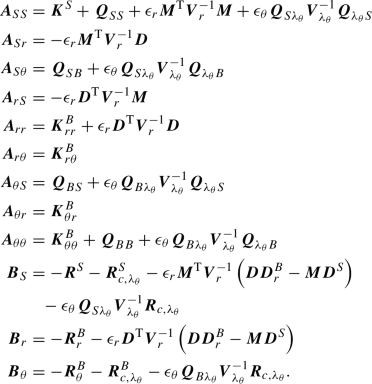(75)

## Examples

The following numerical examples are set up using the beam finite element pre-processor MeshPy  and are simulated with our in-house parallel multi-physics research code BACI .

### Single element moment test

The first problem setup is depicted in Fig. 5. A straight beam is embedded inside a solid cube ($$E=1 {\hbox {N}/\hbox {m}^{2}}$$, $$\nu =0$$) and the beam is loaded with a distributed torsion moment in $$\underline{\varvec{{e}}}_1$$ direction, which is constant along the beam centerline. This example is used to investigate how a moment on a beam is transferred to solid nodal forces. The cube is modeled with a single eight-noded hexahedral element and all solid degrees of freedom are fixed. A single Simo–Reissner beam finite element is used to discretize the beam. No Dirichlet boundary conditions are applied on the beam and the coupling between the beam and the solid is realized with our novel mortar-type BTS-FULL method. Thus, the only interaction between the beam and the solid is the transfer of the external moment. The resulting nodal reaction forces for the different solid triad definitions introduced in Sect.5 are depicted in Fig. 6. Therein, the results for the $${\hbox {STR}-\text {POL}}$$, $${\hbox {STR}-{\text {AVG}}}$$ and $${\hbox {STR}-\text {ORT}}$$ variants, cf. Fig. 6a, 6d and 6e, match up to machine precision. In general, however, the solid coupling reaction forces may differ for different definitions of the solid triad, as visible for the variants $${\hbox {STR}-{\text {DIR}_{2/3}}}$$ in Fig. 6 and c. This observation can be explained by the fact that the representation of a moment via nodal forces is non-unique, i.e. there is an infinite number of possible force pair combinations to achieve this. However, from a mechanical point of view, the force pairs resulting from the $${\hbox {STR}-\text {POL}}$$, $${\hbox {STR}-{\text {AVG}}}$$ and $${\hbox {STR}-\text {ORT}}$$ variants seem more natural than the ones for the $${\hbox {STR}-{\text {DIR}_{2/3}}}$$ variants. Moreover, the former three variants result in the (unique) force pair solution if the moment is applied as a constant shear stress on the beam surface, cf. Sect. 2. Additionally, it can be observed for the $${\hbox {STR}-{\text {DIR}_{2/3}}}$$ variants that the choice which local solid direction is coupled to the solid triad drastically affects the result for the nodal forces.

### Shear test

The next elementary test case is illustrated in Fig. 7. The problem geometry is the same as in the previous example. The cube (side length $$h = 1{\hbox {m}}$$) is fixed at two bottom corner points to constrain all rigid body modes. A constant surface load $$\tau = 0.001{\hbox {N}/\hbox {mm}^2}$$ is applied to the surfaces of the cube, as depicted in Fig. 7. No boundary conditions are applied to the beam. This problem illustrates how the specific solid triads affect the shear stiffness of the solid element and will be studied in two steps.

In a first step, we want to investigate the impact of the local stiffening effect the beam cross-section has on the surrounding solid material. To do so, a reference solution is created by applying a full 2D-3D beam-to-solid coupling scheme, i.e. the coupling conditions are enforced on the beam surface, cf.  Appendices 2 and 3. For comparison purposes, a variant of this problem is simulated without the embedded beam, i.e. the pure solid shear problem. Figure 8 illustrates the shear stress in the solid, with and without the embedded beam, for an exemplary beam radius $$r=0.1{\hbox {m}}$$. As expected, the solution is uniform in the entire solid volume for the pure solid variant. In the 2D-3D beam-to-solid coupling variant, the embedded beam affects the solid stress and displacement fields. The overall displacement of the solid is smaller than for the variant without a beam, thus demonstrating the stiffening effects of the beam cross-section. In agreement with our fundamental modeling assumption of overlapping beam and solid domains (see Sect. 2), the solid shear stress inside the beam domain is zero. Outside of the beam domain, the solid shear stress field shows slight fluctuations due to the local constraints enforcing the 2D-3D coupling at the beam surface. However, close to the boundaries of the cube, these fluctuations become negligible and the shear stress field is quite homogeneous and therefore very similar to the pure solid shear problem.

In a second step, this problem is simulated with one single solid finite element to investigate potential shear locking effects. The coupling between beam and solid is now realized with our novel BTS-FULL method and a rotational penalty parameter of $$\epsilon _{\theta }= 100{\hbox {Nm}/\hbox {m}}$$. In Fig. 9, the deformed solid element and the resulting coupling reaction forces on the solid nodes are depicted for the different solid triad definitions and again for the problem without embedded beam. Due to the orthogonality constraints in the $${\hbox {STR}-\text {ORT}}$$ variant, no shear mode remains in the solid finite element, i.e. it is rigid with respect to shear deformations (in fact, small deformations can be observed due to the penalty regularization). In this example, all other solid triad definitions result in a solid displacement field matching the variant without embedded beam up to machine precision. Table 2 states the rigid body rotation angle $$\psi _{B}$$ of the beam for the different solid triad variants. The rotation angle $$\psi _{B}$$ of the beam depends on the employed solid triad variant. With the $${\hbox {STR}-{\text {DIR}_{2}}}$$ variant the beam does not rotate at all since the orientation of the local solid material fiber does not change. The $${\hbox {STR}-{\text {DIR}_{3}}}$$ variant, on the other hand, results in the largest rotation of the beam, since the solid triad is coupled to the solid material fiber which undergoes the largest orientation change. Although they are not identical up to machine precision, the $${\hbox {STR}-\text {POL}}$$ and $${\hbox {STR}-{\text {AVG}}}$$ variants lead to very similar results for the rotation of the beam, i.e. roughly an average of the $${\hbox {STR}-{\text {DIR}_{2}}}$$ and $${\hbox {STR}-{\text {DIR}_{3}}}$$ variants.

The results show that the presented solid triads lead to either no shear stiffening effects in the solid ($${\hbox {STR}-\text {POL}}$$, $${\hbox {STR}-{\text {DIR}_{2,3}}}$$ and $${\hbox {STR}-{\text {AVG}}}$$) or to severe locking resulting in a complete constraining of all shear modes ($${\hbox {STR}-\text {ORT}}$$). To assess which variant resembles best the resolved 2D-3D coupling scheme, the relative $$L_2$$-displacement error

\begin{aligned} \left\| e\right\| _{L2,\text {rel}}= \frac{\sqrt{\int _{\varOmega _{S,0}}{ \left\| \underline{\varvec{{u}}}^S_h- {\underline{\varvec{{u}}}^S_{\text {ref}}}\right\| ^2 \,\mathrm d V_0}\,}}{\sqrt{\int _{\varOmega _{S,0}}{ \left\| {\underline{\varvec{{u}}}^S_{\text {ref}}}\right\| ^2 \,\mathrm d V_0}\,}} \end{aligned}
(76)

is compared. In the results presented in the following, the reference solution is the solution obtained with a fine solid mesh and a 2D-3D coupling. Figure 10 illustrates $$\left\| e\right\| _{L2,\text {rel}}$$ for different beam diameter to solid cube length ratios d/h. The relative error for the $${\hbox {STR}-\text {ORT}}$$ variant is almost constant 1 for all beam diameters ratios, i.e. even for beam cross-section sizes similar to the cube dimensions a full constraining of all shear modes does not accurately describe the physical coupling. For all other variants the behavior of the relative error is the same, since none of them constrain the shear deformation mode in the solid, i.e. the beam cross-section s rotate with the solid without constraining it. For small ratios of beam radius to solid cube length the error is close to zero. For larger ratios of beam radius to solid cube length, the error increases as there is a real physical stiffening effect due to the embedded beam cross-section in the 2D-3D problem that is not captured by the 1D-3D coupling schemes. However, in the entire range of practically relevant solid mesh sizes (relative to the beam cross-section size) as illustrated in Fig. 10, the solid triad variants that do not constrain the in-plane deformation of the solid result in a better approximation of the physical system behavior as compared to the $${\hbox {STR}-\text {ORT}}$$ triad.

In this example, multiple fibers are placed inside a solid cube, cf. Fig. 11. The solid cube has the dimensions $$1{\hbox {m}}\times 1{\hbox {m}}\times 1{\hbox {m}}$$ and consists of a hyperelastic Saint Venant–Kirchhoff material model ($$E=1{\hbox {N}/\hbox {m}^2}$$, $$\nu =0.0$$). Embedded inside the solid cube are $$5 \times 5$$ fibers with a radius of $$0.0125{\hbox {m}}$$. All fibers point in $$\underline{\varvec{{e}}}_3$$ direction. The solid is fixed in $$\underline{\varvec{{e}}}_2$$ direction at the left boundary, and loaded with two equilibrating shear loads ($$\tau =0.01{\hbox {N}/\hbox {m}^2}$$) at the left and right boundary. To constrain the remaining rigid body mode, the lower left corner point is fixed in $$\underline{\varvec{{e}}}_3$$ axis. The fibers are coupled to the solid via the BTS-FULL method and no additional boundary conditions act on the fibers. The cube is meshed with $$7\times 7\times 1$$ solid hex8 elements, and each fiber is represented by a single Simo–Reissner beam finite element. The penalty parameters for the BTS-FULL method are $$\epsilon _r= 100{\hbox {N}/\hbox {m}^2}$$ and $$\epsilon _{\theta }= 100{\hbox {Nm}/\hbox {m}}$$. In this example, the results obtained with the BTS-FULL method and different solid triads will be compared with a spatially converged reference solution, where the coupling between the beam surfaces and solid volume is discretized in a surface-to-volume (2D-3D) manner, i.e. the beam surface instead of the centerline is fixed to the solid, cf. Appendices 2 and 3.

The resulting shear stresses are visualized in Fig. 12. In the full 2D-3D model, there are stress concentrations at the interface between the beam surfaces and the solid. It is important to point out that the BTS-FULL method (1D-3D), is not able to capture these stress concentrations, regardless of the employed solid triad. However, this has not been the intention of the BTS-FULL method in the first place, but instead we want to make sure that the far field stress in the solid is represented accurately. Figure 12 illustrates the shear stress results obtained with the $${\hbox {STR}-\text {POL}}$$, $${\hbox {STR}-{\text {DIR}_{2/3}}}$$ and $${\hbox {STR}-{\text {AVG}}}$$ solid triads. In the reference solution the in-plane shear stress is positive at the top and bottom of the cube and negative in the middle. The results obtained with the $${\hbox {STR}-\text {POL}}$$, $${\hbox {STR}-{\text {DIR}_{2/3}}}$$ and $${\hbox {STR}-{\text {AVG}}}$$ solid triads are similar to the ones obtained with 2D-3D coupling. However, the results with the $${\hbox {STR}-\text {ORT}}$$ solid triads clearly exhibit drastic shear locking effects due to the (over-) constraining of orthogonal solid directions. Table 3 provides the displacement at the top right corner of the cube for the 2D-3D reference solution and different types of solid triad fields, as well as the relative error. The error for the $${\hbox {STR}-\text {ORT}}$$ solid triad is six times larger than for all other solid triads. This again illustrates the unwanted locking effects introduced by the $${\hbox {STR}-\text {ORT}}$$ solid triads variant.

At this point a short recap of the first three examples for each of the investigated solid triad constructions is given to summarize their applicability in the context of our BTS-FULL method:

$${\hbox {STR}-\text {POL}}$$:

All basic consistency tests are fulfilled by this variant. However, due to the computational complexity of the polar decomposition in 3D, cf. Sect. 5.2, this variant is not used in the remaining examples presented in this contribution.

$${\hbox {STR}-{\text {DIR}_{2/3}}}$$:

The examples show that the (arbitrary) choice of the solid material direction for the construction of the solid triad can have a considerable effect on the results. Therefore, these variants will not be employed in the following. However, for comparison purposes they will be included in the spatial convergence example, cf. Sect. 7.5.

$${\hbox {STR}-{\text {AVG}}}$$:

All basic consistency tests are fulfilled by the averaged solid triad and the results are very close to the ones obtained via the $${\hbox {STR}-\text {POL}}$$ variant, while being less expensive from a computational point of view. This variant is used in the remaining examples of this contribution.

$${\hbox {STR}-\text {ORT}}$$:

This variant leads to considerable shear locking in the range of coarse solid mesh resolutions, which is exactly the range of interest for the proposed 1D-3D coupling schemes. Therefore, this variant will not be used in the remainder of this contribution.

### Transfer of constant torque

This example serves as a consistency test for the BTS-FULL method and its ability to transfer a constant torque. It is an extension of the constant stress transfer problem for the BTS-TRANS method previously presented in . The example is inspired by classical patch tests, which are well-established tools to investigate the consistency of finite element formulations . The constant torque test is depicted in Fig. 13. It consists of a solid block $$\varOmega _S$$ with two embedded beams $$\varOmega _{B1}$$ and $$\varOmega _{B2}$$. The two beams occupy the same spatial position. The solid is fixed at the lower surface and no external loads are applied. One beam is loaded with a torsion load $$\underline{\varvec{{m}}}$$, and the other beam with a torsion load $$-\underline{\varvec{{m}}}$$, both acting along their axial direction. The magnitude of the torsion load is $$10{\hbox {Nm}/\hbox {m}}$$. Based on the space-continuous problem description, the opposing loads on the two beams cancel out each other, and in sum the two beams transfer no loads to the solid. This gives the trivial solution $$\underline{\varvec{{u}}}_S= \underline{\varvec{{0}}}$$ for the displacement field in the solid, cf. , and a constant solution for the beam rotations along their axis. In this test it shall be verified that this solution can also be represented in the spatially discretized setting using an arbitrarily coarse discretization. Both beams are coupled to the solid via the BTS-FULL method. There is no direct interaction between the two beams, but all interactions are transferred through the solid domain.

The geometry and material parameters are taken from . The dimensions of the solid block are $$1{\hbox {m}}\times 1{\hbox {m}}\times 2{\hbox {m}}$$ and a Saint Venant–Kirchhoff material model ($$E = 10{\hbox {N}/\hbox {m}^2}$$, $$\nu = 0.3$$) is employed. The block is discretized with $$4\times 4 \times 7$$ eight-noded, first-order hexahedral elements. The circular cross-section s of the two beams have a radius of $$0.05{\hbox {m}}$$, and the beam material parameters are $$E=100{\hbox {N}/\hbox {m}^2}$$ and $$\nu =0$$. The beams B1 and B2 are discretized with 5 and 7 Simo–Reissner beam finite elements, respectively. This results in a non-matching discretization between the two beams as well as between the beams and the solid. Coupling between the beams and the solid is realized with a linear interpolation of both the translational and rotational Lagrange multipliers. The $${\hbox {STR}-{\text {AVG}}}$$ solid triads are employed in this example, cf. Sect. 5.3.2. The penalty parameters are $$\epsilon _r= 100{\hbox {N}/\hbox {m}^2}$$ and $$\epsilon _{\theta }= 100{\hbox {Nm}/\hbox {m}}$$.

Figure 14 illustrates the results of this test. The stress in the solid and the curvature in the beam are indeed zero up to machine precision, thus matching the expected analytical solution.

This example illustrates the ability of the BTS-FULL method to exactly represent a constant torsion state along the beam and the consistency of the coupling terms despite the fact that arbitrary non-matching meshes are involved.

### Spatial convergence

This numerical example investigates the spatial convergence properties of the BTS-FULL method under uniform mesh refinement. The problem is depicted in Fig. 15. It consists of a solid block with the dimensions $$5{\hbox {m}}\times 1{\hbox {m}}\times 1{\hbox {m}}$$ and a Saint Venant–Kirchhoff material model ($$E = 10{\hbox {N}/\hbox {m}^2}$$, $$\nu = 0$$). A beam (cross-section radius $$0.125{\hbox {m}}$$, $$L=5{\hbox {m}}$$, $$E=300{\hbox {N}/\hbox {m}^2}$$, $$\nu =0$$) is embedded inside the solid block. No external loads or Dirichlet boundary conditions are applied to the beam, i.e. homogeneous Neumann boundary conditions at both ends. The right end of of the block is loaded with a shear stress $$\underline{\varvec{{\tau }}}$$. The shear stress at point $$\underline{\varvec{{p}}} = L \underline{\varvec{{e}}}_1+ y \underline{\varvec{{e}}}_2+ z \underline{\varvec{{e}}}_3$$ reads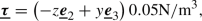(77)

thus resulting in a total torque of $$1.65885\cdot 10^{-2}{\hbox {Nm}}$$. This example can be interpreted as an adapted version of the spatial convergence problem in  to verify the scenario of rotational coupling. A similar problem is also investigated in . The spatial convergence behavior of the BTS-FULL method will be analyzed with respect to a spatially converged reference solution obtained with a 2D-3D coupling discretization, as described in Sect. 2. To compare the results, the $$L_2$$ displacement error in the solid is calculated via

\begin{aligned} \left\| e\right\| _{L2}= \frac{1}{V_0} \sqrt{\int _{\varOmega _{S,0}}{ \left\| \underline{\varvec{{u}}}^S_h- {\underline{\varvec{{u}}}^S_{\text {ref}}}\right\| ^2 \,\mathrm d V_0}\,}. \end{aligned}
(78)

Here, $$V_0=1{\hbox {m}^3}$$ is the solid volume in the reference configuration. It should be pointed out that the 2D-3D coupling problem does not have the same analytical solution as the BTS-FULL problem, because the 1D-3D coupling results in a singularity in the analytical solution, cf. Sect. 2.1. Therefore, spatial convergence of the BTS-FULL method towards the reference solution is not expected all the way towards the asymptotic limit of arbitrarily small solid element sizes, but only in the practically relevant regime of solid mesh sizes that are larger than the beam cross-section radius. In this regime, the singularity, i.e. the difference between the 1D-3D and 2D-3D models can not be fully resolved by the finite element solution space. This fact can be exploited to obtain reasonably accurate results with our BTS-FULL (i.e. 1D-3D) method for the envisioned applications and practically relevant mesh resolutions.

The solution to the presented problem has a point symmetry around the $$\underline{\varvec{{e}}}_1$$ axis. Therefore, the $${\hbox {STR}-\text {POL}}$$ and $${\hbox {STR}-{\text {AVG}}}$$ solid triad variants coincide and give the same numerical results up to machine precision. Similarly, the results obtained with the $${\hbox {STR}-{\text {DIR}_{1/2}}}$$ variants match up to machine precision. Figure 16 shows the convergence plot for different types of solid triads as well as for the 2D-3D coupling approach. For coarse discretizations an excellent convergence behavior can be observed for the BTS-FULL variants, slightly below the convergence rate of the reference 2D-3D method, but with a significantly reduced computational cost. All BTS-FULL convergence plots exhibit a kink at a certain solid mesh resolution: the $${\hbox {STR}-{\text {DIR}_{1/2}}}$$ variants at around $$h_{\text {solid}}= 0.07{\hbox {m}}$$, and the $${\hbox {STR}-\text {POL}}$$ and $${\hbox {STR}-{\text {AVG}}}$$ variants at around $$h_{\text {solid}}= 0.06{\hbox {m}}$$. Figuratively speaking, the difference between the 1D-3D and 2D-3D coupling model becomes dominant at the kink position, since the solid element size to beam cross-section diameter ratio becomes smaller. Nevertheless, the kink only occurs when the solid element size is already smaller than the cross-section radius, which is far away from the envisioned geometric relations for the BTS-FULL method anyways. The results confirm that for solid element sizes larger than the beam cross-section diameter, i.e. our desired and practically relevant discretization case, the results obtained with the BTS-FULL method (1D-3D) exhibit excellent spatial convergence properties and thus give a very good approximation of the 2D-3D coupling problem.

### Plane cantilever bending

In this example we consider a cantilever structure modeled as a solid continuum subject to a moment load. The problem is illustrated in Fig. 17a. The cantilever has the dimensions $$5{\hbox {m}}\times 1{\hbox {m}}$$ and consists of a Saint Venant–Kirchhoff material ($$E = 10{\hbox {N}/\hbox {m}^2}$$, $$\nu = 0$$). At the left boundary all displacement components are fully constrained. A moment load $$M=0.0290888{\hbox {Nm}}$$ acts on the cantilever at the material point $$\underline{\varvec{{X}}}_M={[4.5, 0]^{\mathrm T}}{\hbox {m}}$$. This moment is chosen such that, if the cantilever were modeled using 1D beam theory, it should bend exactly to a quarter circle, due to a pure bending deformation in the region between the Dirichlet boundary and the applied moment. Directly imposing a conservative moment load on a solid, i.e. a Boltzmann continuum, which exhibits no rotational degrees of freedom is a non-trivial task. Standard approaches would require to model the moment as a (deformation-dependent) load/traction field distributed across an arbitrarily chosen sub-volume of the solid. In this example we impose the external moment on the solid structure by defining a solid triad ($${\hbox {STR}-{\text {AVG}}}$$) at the application point of the moment. The nodal external forces effectively acting on the solid are obtained by projecting the moment to the solid finite element space via the discrete version of (52). The cantilever is discretized with $$25\times 5$$ plane four-noded, first-order quadrilateral elements. In Fig. 17b the deformed cantilever is illustrated. The global displacement behavior is as expected, i.e. the cantilever bends to a quarter circle. Of course, the local strain state close to the point where the external moment is applied is not meaningful in a continuum mechanics sense, since we impose a singular moment at that point. However, according to Saint Venant’s principle, a linear stress distribution across the beam height, as expected for the pure bending of a slender beam-like structure, can be observed at a sufficient distance from the point where the moment is induced. This example illustrates that the presented rotational coupling approach is not limited to the coupling of beam cross-section orientations, but can also be used as a stand-alone feature to impose moments onto a solid domain in a variationally consistently manner. It should be pointed out that this example has only been carried out in 2D for reasons of simplicity, while the illustrated capability is available in 3D problems, too.

### Plate with embedded beam

In this example a beam is only partially embedded inside a solid plate and loaded with a tip force. Two different geometry variants of the embedded beam are considered, cf. Fig. 18. In variant A the embedded part of the beam has the shape of a quarter circle, while it is straight in variant B. The plate has the dimensions $$1{\hbox {m}}\times 1{\hbox {m}}\times 0.1{\hbox {m}}$$ and consists of a Saint Venant–Kirchhoff material ($$E = 1{\hbox {N}/\hbox {m}^2}$$, $$\nu = 0.3$$). The embedded Simo–Reissner beam has a cross-section radius $$r= 0.025{\hbox {m}}$$ and the material parameters are $$E = 100{\hbox {N}/\hbox {m}^2}$$ and $$\nu = 0$$. In both variants the beam is loaded with a tip load $$\underline{\varvec{{F}}} =$$-$$0.0001{\hbox {N}} \,\underline{\varvec{{e}}}_3$$ at the end that sticks out of the solid domain. The solid plate is fully clamped at the left and at the bottom.

The coupling of beam and solid is realized with our novel BTS-FULL method and compared to the BTS-TRANS method from , i.e. the one without rotational coupling. First-order Lagrange polynomials are employed to discretize the translational and rotational Lagrange multipliers. The penalty parameters are $$\epsilon _r= 100{\hbox {N}/\hbox {m}^2}$$ and $$\epsilon _{\theta }= 100{\hbox {Nm}/\hbox {m}}$$. The solid plate is modeled with $$1\times 10\times 10$$ eight-noded solid-shell elements [10, 69], while the entire beam is discretized with six Simo–Reissner beam finite elements. The resulting global finite element model has 807 degrees of freedom. A full 3D model, also resolving the beam with three-dimensional solid finite elements and consisting of 90, 190 second-order tetrahedra (tet10) elements, serves as a comparison. The discretization of the full 3D model has been chosen such that mesh convergence is guaranteed. Consequently, the full 3D model consists of 270, 570 degrees of freedom.

The results for variant A are shown in Fig. 19. It can be seen that the the full 3D model and the new BTS-FULL method exhibit the same overall behavior, while the beam experiences much larger deformations and the solid smaller ones in the BTS-TRANS model without rotational coupling. This is due to the fact that in the full 3D problem a considerable portion of the external load is transferred from the beam to the solid via shear stresses on the beam surface, which are represented by moments in the reduced-dimensional model. Only the new BTS-FULL method is able to capture these coupling moments. Figure 20 shows the results for variant B. In this case a solution for the purely translational BTS-TRANS method (i.e. only centerline position coupling) does not even exist within a quasi-static framework, since the beam has an unconstrained rigid body rotation mode around its axis of the embedded part. Again, the displacement results of the full 3D problem and the BTS-FULL model are very close to each other. A more detailed comparison of the different variants is given in Fig. 21. Therein, the displacements along the curve indicated in Fig. 18 are visualized. Now it also becomes clear quantitatively that the displacement results obtained with the BTS-FULL method are very close to the ones obtained with the full 3D problem. Considering that the former reduces the number of degrees of freedom by a factor of about 330 as compared with the latter, this is a remarkable result and showcases the efficiency of the new BTS-FULL method for challenging applications.

### Twisted plate

In this final example we consider a plate, with complex, spatially distributed fiber reinforcements in 3D, cf. Fig. 22. The plate has the dimensions $$1{\hbox {m}}\times 3.5{\hbox {m}}\times 0.1{\hbox {m}}$$ and consists of a Neo-Hookean material ($$E = 1{\hbox {N}/\hbox {m}^2}$$, $$\nu = 0.3$$). The plate is fully clamped at the left face. The right face of the plate is rotated around the $$\underline{\varvec{{e}}}_2$$ axis with the rotation angle $$\phi = [0, 2 \pi ]$$, i.e. the plate is twisted along the $$\underline{\varvec{{e}}}_2$$ axis. Different shapes of fibers are embedded in the plate: semicircles with a radius of $$0.25{\hbox {m}}$$ and straight lines with a length of $$0.6{\hbox {m}}$$. The fiber semicircles are rotated by $$\pm 15^{\circ }$$ with respect to the $$\underline{\varvec{{e}}}_2$$ axis to make the example more challenging and represent general 3D fiber-solid element intersection scenarios. The embedded fibers have a cross-section radius $$r=0.01{\hbox {m}}$$ and the material parameters are $$E = 400{\hbox {N}/\hbox {m}^2}$$ and $$\nu = 0$$. The coupling of fibers and solid is realized with the BTS-FULL method ($${\hbox {STR}-{\text {AVG}}}$$ solid triad, $$\epsilon _r= 100{\hbox {N}/\hbox {m}^2}$$ and $$\epsilon _{\theta }= 100{\hbox {Nm}/\hbox {m}}$$). First-order Lagrange polynomials are employed to discretize the translational and rotational Lagrange multipliers. The solid plate is modeled with $$10\times 35\times 2$$ eight-noded solid-shell elements, while each fiber is discretized with four Simo–Reissner beam finite elements, thus resulting in a total of 92 beam finite elements. The displacement controlled twisting deformation of the plate is applied within 100 quasi-static load steps. At this point it should be mentioned that this example could not be solved with the BTS-TRANS method, since the rigid body rotation modes of the straight fibers lead to a non-converging Newton–Raphson algorithm in the very first load step. This underlines the advantages of the mechanically consistent coupling provided by the BTS-FULL method.

Figure 23 illustrates the deformed structure at different load steps. Until load step 75, the reinforced plate exhibits a more or less homogeneous twist along the $$\underline{\varvec{{e}}}_2$$ axis. From load step 75 to load step 100, the reinforced plate folds around the $$\underline{\varvec{{e}}}_2$$ axis. To assess the non-linear behavior of this structure and evaluate the global impact of the fiber-reinforcements, the fiber-reinforced plate is compared to a simple plate (same material) without any fibers. Fig. 24 depicts the reaction moment $$M_2$$ around the $$\underline{\varvec{{e}}}_2$$ axis at the fully clamped surface of the plate with and without fiber-reinforcements. Until load step 70, the structures behave similarly. However, as expected the fiber-reinforcements lead to an increased reaction moment for the same twist angle $$\phi$$, i.e. to a stiffer structural response. Both structures exhibit a limit point with an unstable post-critical solution, i.e. the structures would collapse if the twist is applied in a load-controlled manner. The fiber-reinforcements affect the critical point of the structure such that the instability occurs at a smaller twist angle and the critical moment is increased. This illustrates the complex influences that fiber-reinforcements may have on the global non-linear behavior of a structure.

Figure 25 illustrates the final configuration and shows a close-up view of the deformed embedded fibers. The maximum normal stresses in the fibers resulting from axial and bending deformations can be estimated for this example as $$\approx 15{\hbox {N}/\hbox {m}^2}$$ and $$\approx 26{\hbox {N}/\hbox {m}^2}$$ (not visualized in the figure), respectively. In the solid the maximum principal Cauchy stress is $$0.578{\hbox {N}/\hbox {m}^2}$$ (not visualized in the figure). As expected, the stresses in the stiff fibers are much larger than in the relatively soft solid matrix. To further investigate the influences of the different deformation modes of the fibers, Fig. 26 depicts the tension, shear, torsion and bending contributions to the total internal elastic energy of the fibers over the course of the simulation. In the first few load steps, the main contributors to the internal elastic energy of the system are bending and torsion deformations, cf. right part of Fig. 26. This can be attributed to the fact that in the beginning of the simulation the deformations of the plate mainly take place in in $$\underline{\varvec{{e}}}_3$$ direction, which predominantly causes bending and torsion deformations of the fibers. As the plate is twisted further, geometrically non-linear effects materialize especially on the outer edges of the plate. The edges form helix like curves. Due to the constrained displacements in $$\underline{\varvec{{e}}}_3$$ direction at the clamped surfaces, the outside edges of the plate are stretched in $$\underline{\varvec{{e}}}_3$$ direction. This causes axial tension in the fiber semicircles at the outside. Starting at approximately load step 25, the main contribution to the internal elastic fiber energy comes from axial deformations. In the post-buckling state, the bending deformation of the plate, and therefore also of the fibers increases. This causes an increase in the internal elastic bending and torsion energy of the fibers. Moreover, shear deformations only have a minor contribution to the total internal energy of the fibers, which is expected due to the slenderness of the embedded fibers, thus motivating a future use of the BTS-FULL method in combination with shear stiff Kirchhoff–Love beam theories [36, 37].

The considerable contributions of bending and torsional energy to the internal elastic energy of the fibers demonstrates the importance of consistently representing these modes and coupling them to the background solid material as done by our proposed BTS-FULL scheme. For this example, this would not be the case if simplified models for the fibers (e.g. modeled as strings without bending stiffness) or for the fiber-solid coupling (e.g. BTS-TRANS) were applied.

This example also showcases the maturity of the implemented BTS-FULL method from an algorithmic point of view. The chosen solid mesh, in combination with the tilted fiber semicircles results in complex 3D intersections between the beam finite elements and the solid finite elements, thus illustrating the robustness of the employed numerical integration algorithm. As a final example, a more complex model of a fiber-reinforced plate is considered. Therein, the dimensions of the plate are repeated 5 times in $$\underline{\varvec{{e}}}_1$$ and $$\underline{\varvec{{e}}}_2$$ direction and 3 times in $$\underline{\varvec{{e}}}_3$$ direction. The pattern and size of the fiber-reinforcements is similar to the one illustrated in Fig. 22, however, in this case there are 3 layers of fiber-reinforcements over the thickness of the plate. This results in a total of approximately 53, 000 solid finite elements and 1, 800 fibers with 4 beam finite elements each, i.e. the problem size is scaled by a factor of approximately 75 compared to the previously considered plate. The deformed configuration of the plate is visualized in Fig. 27 . This further illustrates the robustness and scalability of the presented BTS-FULL method for large-scale problems.

## Conclusion

In this work we have proposed a 1D-3D coupling method to consistently embed 1D Cosserat beams into 3D Boltzmann continua (solids). Six constraint equations act on each point along the Cosserat beam centerline, namely three translational constraints and three rotational constraints, thus resulting in a full, mechanically consistent coupling between the 1D beams and the 3D continuum. Deriving the full 1D-3D coupling on the beam centerline from a 2D-3D coupling on the beam surface via a Taylor series expansion of the solid displacement field would require to fully couple the deformed solid directors with the undeformable beam cross-section triad. It is demonstrated that such an approach, which suppresses all in-plane deformation modes of the solid at the coupling point, might result in severe locking effects in the practically relevant regime of relatively coarse solid mesh sizes. Therefore, a suitable triad field has to be defined in the 3D Boltzmann continuum that only represents solid material directions in an average sense without constraining them. It has been shown that the rotational part of the polar decomposition of the (in-plane projection of the) solid deformation gradient is a natural choice, since it represents the average orientation of material directions of the 3D continuum in a $$L_2$$-optimal manner. Additionally, several other solid triad definitions have been presented, which allow for a more efficient numerical evaluation. Furthermore, existing Lagrange multiplier-based coupling methods for the translational degrees of freedom have been extended for the coupling of rotational degrees of freedom, all within the theory of large rotations. The coupling equations have been discretized using a mortar-type approach and enforced using a regularized weighted penalty method. Based on elementary numerical test cases, it has been demonstrated that a consistent spatial convergence behavior can be achieved and potential locking effects can be avoided, if the proposed BTS-FULL scheme is combined with a suitable solid triad definition. Furthermore, numerical experiments have been conducted to show the applicability of the proposed method to real-life engineering applications.

Future work will focus on the extension of the proposed beam-to-solid volume coupling approach to beam-to-solid surface coupling as well as to beam-to-solid surface contact. Especially in the latter case, the proposed rotational coupling constraints are essential to capture effects such as frictional contact between beam and solid. Another topic of interest for future research is the combination of 1D-3D and 2D-3D coupling within a unified beam-to-solid coupling approach. This would allow to use 2D-3D coupling along with a refined solid mesh only in domains where high resolution of solid stress fields is of interest, and using the proposed, highly efficient 1D-3D coupling approach in the remaining problem domain. Moreover, also a combination of the developed schemes with concepts allowing for a consistent coupling of the beam ends with the solid domain, cf. , are considered as promising future research direction.

## References

1. Agarwal BD, Broutman LJ, Chandrashekhara K (2017) Analysis and performance of fiber composites, 4th edn. John Wiley & Sons

2. Alberts B, Johnson A, Lewis J, Raff M, Roberts K, Walter P (2008) Molecular biology of the cell, 5th edn. Garland Science, NY

3. Argyris J (1982) An excursion into large rotations. Comput Methods Appl Mech Eng 32(1):85–155

4. BACI: A Comprehensive Multi-Physics Simulation Framework. https://baci.pages.gitlab.lrz.de/website (2021)

5. Bauchau OA, Han S, Mikkola A, Matikainen MK (2014) Comparison of the absolute nodal coordinate and geometrically exact formulations for beams. Multibody Syst Dynam 32(1):67–85

6. Béchet É, Moës N, Wohlmuth B (2009) A stable Lagrange multiplier space for stiff interface conditions within the extended finite element method. Int J Numer Methods Eng 78(8):931–954

7. Ben Belgacem F (1999) The mortar finite element method with Lagrange multipliers. Numer Math 84(2):173–197

8. Betsch P, Menzel A, Stein E (1998) On the parametrization of finite rotations in computational mechanics: a classification of concepts with application to smooth shells. Comput Methods Appl Mech Eng 155(3):273–305

9. Betsch P, Steinmann P (2002) Frame-indifferent beam finite elements based upon the geometrically exact beam theory. Int J Numer Methods Eng 54(12):1775–1788

10. Bischoff M, Ramm E (1997) Shear deformable shell elements for large strains and rotations. Int J Numer Methods Eng 40(23):4427–4449

11. Boffi D, Brezzi F, Fortin M (2013) Mixed finite element methods and applications, 1st edn. Springer, NY

12. Cardona A, Geradin M (1988) A beam finite element non-linear theory with finite rotations. Int J Numer Methods Eng 26(11):2403–2438

13. Chang TY, Taniguchi H, Chen WF (1987) Nonlinear finite element analysis of reinforced concrete panels. J Struct Eng 113(1):122–140

14. Crisfield MA, Jelenić G (1999) Objectivity of strain measures in the geometrically exact three-dimensional beam theory and its finite-element implementation. Proc R Soc London A 455(1983):1125–1147

15. Dolbow J, Harari I (2009) An efficient finite element method for embedded interface problems. Int J Numer Methods Eng 78(2):229–252

16. Durville D (2007) Finite element simulation of textile materials at mesoscopic scale. In: Finite element modelling of textiles and textile composites

17. Elwi AE, Hrudey TM (1989) Finite element model for curved embedded reinforcement. J Eng Mech 115(4):740–754

18. Farah P, Popp A, Wall WA (2015) Segment-based versus element-based integration for mortar methods in computational contact mechanics. Comput Mech 55(1):209–228

19. Favata A (2012) On the Kelvin problem. J Elast 109(2):189–204

20. Gomes HM, Awruch AM (2001) Some aspects on three-dimensional numerical modelling of reinforced concrete structures using the finite element method. Adv Eng Softw 32(4):257–277

21. Grill MJ, Eichinger JF, Koban J, Meier C, Lieleg O, Wall WA (2021) A novel modeling and simulation approach for the hindered mobility of charged particles in biological hydrogels. Preprint, https://arxiv.org/abs/2101.06756

22. Hagmeyer N, Mayr M, Steinbrecher I, Popp A (2021) Fluid-beam interaction: Capturing the effect of embedded slender bodies on global fluid flow and vice versa. Preprint, arXiv:2104.09844

23. Hautefeuille M, Annavarapu C, Dolbow JE (2012) Robust imposition of Dirichlet boundary conditions on embedded surfaces. Int J Numer Methods Eng 90(1):40–64

24. Ibrahimbegović A, Frey F, Kožar I (1995) Computational aspects of vector-like parametrization of three-dimensional finite rotations. Int J Numer Methods Eng 38(21):3653–3673

25. Kang J, Kim K, Lim YM, Bolander JE (2014) Modeling of fiber-reinforced cement composites: discrete representation of fiber pullout. Int J Solids Struct 51(10):1970–1979

26. Kerfriden P, Claus S, Mihai I (2020) A mixed-dimensional CutFEM methodology for the simulation of fibre-reinforced composites. Adv Model Simulat Eng Sci 7(1):18

27. Khristenko U, Schuß S, Krüger M, Schmidt F, Wohlmuth B, Hesch C (2021) Multidimensional coupling: a variationally consistent approach to fiber-reinforced materials. Comput Methods Appl Mech Eng 382:113869

28. Korelc J, Wriggers P (2016) Automation of finite element methods. Springer International Publishing, NY

29. Leichner A, Andrä H, Simeon B (2019) A contact algorithm for voxel-based meshes using an implicit boundary representation. Comput Methods Appl Mech Eng 352:276–299

30. Leyendecker S, Betsch P, Steinmann P (2006) Objective energy-momentum conserving integration for the constrained dynamics of geometrically exact beams. Comput Methods Appl Mech Eng 195(19):2313–2333

31. Lieleg O, Claessens MMAE, Bausch AR (2010) Structure and dynamics of cross-linked actin networks. Soft Matter 6:218–225

32. Mattheij P, Gliesche K, Feltin D (1998) Tailored fiber placement—mechanical properties and applications. J Reinforced Plast Compos 17(9):774–786

33. Mattheij P, Gliesche K, Feltin D (2000) 3D reinforced stitched carbon/epoxy laminates made by tailored fibre placement. Compos Part A Appl Sci Manuf 31(6):571–581

34. Meier C, Grill MJ, Wall WA (2021) Generalized section-section interaction potentials in the geometrically exact beam theory. Preprint, arXiv:2105.10032

35. Meier C, Grill MJ, Wall WA, Popp A (2018) Geometrically exact beam elements and smooth contact schemes for the modeling of fiber-based materials and structures. Int J Solids Struct 154:124–146

36. Meier C, Popp A, Wall WA (2014) An objective 3D large deformation finite element formulation for geometrically exact curved Kirchhoff rods. Comp Methods Appl Mech Eng 278:445–478

37. Meier C, Popp A, Wall WA (2015) A locking-free finite element formulation and reduced models for geometrically exact Kirchhoff rods. Comp Methods Appl Mech Eng 290:314–341

38. Meier C, Popp A, Wall WA (2019) Geometrically exact finite element formulations for slender beams: Kirchhoff-Love theory versus Simo-Reissner theory. Arch Comput Methods Eng 26(1):163–243

39. Meier C, Wall WA, Popp A (2017) A unified approach for beam-to-beam contact. Comput Methods Appl Mech Eng 315:972–1010

40. Moës N, Cloirec M, Cartraud P, Remacle JF (2003) A computational approach to handle complex microstructure geometries. Comput Methods Appl Mech Eng 192(28):3163–3177

41. Müller KW, Meier C, Wall WA (2015) Resolution of sub-element length scales in Brownian dynamics simulations of biopolymer networks with geometrically exact beam finite elements. J Comput Phys 303:185–202

42. Pattinson SW, Huber ME, Kim S, Lee J, Grunsfeld S, Roberts R, Dreifus G, Meier C, Liu L, Hogan N, Hart AJ (2019) Additive manufacturing of biomechanically tailored meshes for compliant wearable and implantable devices. Adv Funct Mater 29(32):1901815

43. Phillips DV, Zienkiewicz OC (1976) Finite element non-linear analysis of concrete structures. Proc Instit Civil Eng 61(1):59–88

44. Podio-Guidugli P, Favata A (2014) Elasticity for geotechnicians: a modern exposition of Kelvin, Boussinesq, Flamant, Cerruti, Melan, and Mindlin problems, vol 204. Springer, Cham

45. Popp A, Gee MW, Wall WA (2009) A finite deformation mortar contact formulation using a primal-dual active set strategy. Int J Numer Methods Eng 79(11):1354–1391

46. Popp A, Gitterle M, Gee MW, Wall WA (2010) A dual mortar approach for 3D finite deformation contact with consistent linearization. Int J Numer Methods Eng 83(11):1428–1465

47. Popp A, Wohlmuth BI, Gee MW, Wall WA (2012) Dual quadratic mortar finite element methods for 3D finite deformation contact. SIAM J Sci Comput 34(4):421–446

48. Puso MA (2004) A 3D mortar method for solid mechanics. Int J Numer Methods Eng 59(3):315–336

49. Puso MA, Laursen TA, Solberg J (2008) A segment-to-segment mortar contact method for quadratic elements and large deformations. Comp Methods Appl Mech Eng 197(6):555–566

50. Ranjbaran A (1996) Mathematical formulation of embedded reinforcements in 3D brick elements. Commun Numer Methods Eng 12(12):897–903

51. Reissner E (1972) On one-dimensional finite-strain beam theory: the plane problem. Zeitschrift für angewandte Mathematik und Physik ZAMP 23(5):795–804

52. Romero I (2004) The interpolation of rotations and its application to finite element models of geometrically exact rods. Comput Mech 34(2):121–133

53. Romero I (2008) A comparison of finite elements for nonlinear beams: the absolute nodal coordinate and geometrically exact formulations. Multibody Syst Dynam 20(1):51–68

54. Romero I (2018) Coupling nonlinear beams and continua: variational principles and finite element approximations. Int J Numer Methods Eng 114(11):1192–1212

55. Rüberg T, Cirak F, García Aznar JM (2016) An unstructured immersed finite element method for nonlinear solid mechanics. Adv Model Simul Eng Sci 3(1):28

57. Sanders J, Puso MA (2012) An embedded mesh method for treating overlapping finite element meshes. Int J Numer Methods Eng 91(3):289–305

58. Sanders JD, Laursen TA, Puso MA (2012) A Nitsche embedded mesh method. Comput Mech 49(2):243–257

59. Simo JC, Vu-Quoc L (1986) On the dynamics of flexible beams under large overall motions—The plane case: Part I. J Appl Mech 53(4):849–854

60. Simo JC, Vu-Quoc L (1986) On the dynamics of flexible beams under large overall motions—The plane case: Part II. J Appl Mech 53(4):855–863

61. Simo JC, Vu-Quoc L (1988) On the dynamics in space of rods undergoing large motions—A geometrically exact approach. Comp Methods Appl Mech Eng 66(2):125–161

62. Spurrier RA (1978) Comment on Singularity-free extraction of a quaternion from a direction-cosine matrix. J Spacecraft Rockets 15(4):255–255

63. Steinbrecher I, Mayr M, Grill MJ, Kremheller J, Meier C, Popp A (2020) A mortar-type finite element approach for embedding 1D beams into 3D solid volumes. Comput Mech 66(6):1377–1398

64. Steinbrecher I, Popp A (2021) MeshPy – A general purpose 3D beam finite element input generator. https://compsim.gitlab.io/codes/meshpy

65. Taylor RL, Simo JC, Zienkiewicz OC, Chan ACH (1986) The patch test—A condition for assessing FEM convergence. Int J Numer Methods Eng 22(1):39–62

66. Thomson W (1848) Note on the integration of the equations of equilibrium of an elastic solid. Cambridge Dublin Math J 3:87–89

67. The Trilinos Project Website. https://trilinos.github.io (2021)

68. Tschisgale S, Fröhlich J (2020) An immersed boundary method for the fluid-structure interaction of slender flexible structures in viscous fluid. J Comput Phys 423:109801

69. Vu-Quoc L, Tan XG (2003) Optimal solid shells for non-linear analyses of multilayer composites I Statics. Comput Methods Appl Mech Eng 192(9):975–1016

70. Wiedemann J (2007) Leichtbau: Elemente und Konstruktion, 3rd edn. Springer-Verlag, Berlin Heidelberg

71. Wohlmuth BI (2000) A mortar finite element method using dual spaces for the lagrange multiplier. SIAM J Numer Anal 38(3):989–1012

72. Yang B, Laursen TA, Meng X (2005) Two dimensional mortar contact methods for large deformation frictional sliding. Int J Numer Methods Eng 62(9):1183–1225

## Acknowledgements

Sketches in this work have been created using the Adobe Illustrator plug-in (https://github.com/isteinbrecher/latex2ai).

## Funding

Open Access funding enabled and organized by Projekt DEAL.

## Author information

Authors

### Corresponding author

Correspondence to Ivo Steinbrecher.

### Publisher's Note

Springer Nature remains neutral with regard to jurisdictional claims in published maps and institutional affiliations.

## Appendix

### Proof of $$L_2$$-optimality of $${\hbox {STR}-\text {POL}}$$ triad

In the following, a proof shall be given for (45). First, an angle $$\theta _0 \in [-\pi , \pi ]$$ is defined that represents the orientation of arbitrary in-plane directors in the reference configuration defined to coincide for solid and beam according to $$\underline{\varvec{{g}}}_{S,0}(\theta _0)=\underline{\varvec{{g}}}_{B,0}(\theta _0)=\cos {(\theta _0)} \, \underline{\varvec{{g}}}_{B2,0} +\sin {(\theta _0)} \, \underline{\varvec{{g}}}_{B3,0}$$. The push-forward to the spatial configuration is given by $$\underline{\varvec{{g}}}_{S}= \underline{\varvec{{F}}}^{\underline{\varvec{{n}}}}\, \underline{\varvec{{g}}}_{S,0}(\theta _0)$$ for the solid and $$\underline{\varvec{{g}}}_{B}= \underline{\varvec{{R}}}_B \, \underline{\varvec{{g}}}_{B,0}(\theta _0)$$ for the beam. As stated in Section 5.1, it is a desirable property of the (to be defined) solid triad and therefore also of the beam triad that the base vectors $$\underline{\varvec{{g}}}_{B2}$$ and $$\underline{\varvec{{g}}}_{B3}$$ lie in the $$\underline{\varvec{{n}}}$$-plane, i.e. the plane spanned by the solid base vectors $$\underline{\varvec{{g}}}_{S2}$$ and $$\underline{\varvec{{g}}}_{S3}$$. Thus, in analogy to (42) as stated for the solid, it is assumed that the total rotation of the beam cross-section $$\underline{\varvec{{R}}}_B$$ is split in a multiplicative manner into two successive rotations

\begin{aligned} \underline{\varvec{{R}}}_{B}= \underline{\varvec{{R}}}_{B}^{\text {2D}}\underline{\varvec{{R}}}^{\underline{\varvec{{n}}}}\end{aligned}
(79)

where $$\underline{\varvec{{R}}}^{\underline{\varvec{{n}}}}$$ describes the 3D rotation from $$\underline{\varvec{{\varLambda }}}_{B,0}$$ to $${\bar{\underline{\varvec{{\varLambda }}}}}$$ and $$\underline{\varvec{{R}}}_{B}^{\text {2D}}$$ the quasi-2D rotation from $${\bar{\underline{\varvec{{\varLambda }}}}}$$ to $$\underline{\varvec{{\varLambda }}}_{B}$$. Thus, after push-forward to the intermediate configuration defined by $$\underline{\varvec{{R}}}^{\underline{\varvec{{n}}}}$$ the corresponding directors of solid and beam still coincide:

\begin{aligned} \begin{aligned} \bar{\underline{\varvec{{g}}}}_{S}(\theta _0)=\bar{\underline{\varvec{{g}}}}_{B}(\theta _0)&= \underline{\varvec{{R}}}^{\underline{\varvec{{n}}}}\, {\underline{\varvec{{g}}}}_{B,0}(\theta _0) = \cos {(\theta _0)} \, \bar{\underline{\varvec{{g}}}}_{2} +\sin {(\theta _0)} \, \bar{\underline{\varvec{{g}}}}_{3}. \end{aligned} \end{aligned}
(80)

In the following, the material and spatial principle axes associated with the polar decomposition (43) of the in-plane deformation gradient $$\underline{\varvec{{F}}}^{\text {2D}}$$ are denoted as $$\underline{\varvec{{G}}}_{P2}$$ and $$\underline{\varvec{{G}}}_{P3}$$ as well as $$\underline{\varvec{{g}}}_{P2}=\underline{\varvec{{R}}}_{S}^{\text {2D}}\underline{\varvec{{G}}}_{P2}$$ and $$\underline{\varvec{{g}}}_{P3}=\underline{\varvec{{R}}}_{S}^{\text {2D}}\underline{\varvec{{G}}}_{P3}$$. Since the principle axes $$\underline{\varvec{{G}}}_{Pi}$$ and the orthonormal base vectors $$\bar{\underline{\varvec{{g}}}}_{i}$$ are related by quasi-2D rotations with respect to the normal vector $$\underline{\varvec{{n}}}$$, the directors in (80) can alternatively be stated as

\begin{aligned} \bar{\underline{\varvec{{g}}}}_{S}(\theta _0)=\bar{\underline{\varvec{{g}}}}_{B}(\theta _0) = \cos {({\tilde{\theta }}_0)} \, \underline{\varvec{{G}}}_{P2} +\sin {({\tilde{\theta }}_0)} \, \underline{\varvec{{G}}}_{P3}, \end{aligned}
(81)

where $${\tilde{\theta }}_0={\theta }_0- \theta _{\text {diff}}$$ is defined via the constant offset value $$\theta _{\text {diff}}$$ describing the rotation from $$\bar{\underline{\varvec{{g}}}}_{i}$$ to $$\underline{\varvec{{G}}}_{Pi}$$. The final beam cross-section triad follows from the second (quasi-2D) rotation $$\underline{\varvec{{R}}}_{B}^{\text {2D}}= \underline{\varvec{{R}}}(\theta _B^{\text {2D}} \underline{\varvec{{n}}})$$ from $$\bar{\underline{\varvec{{g}}}}_{i}$$ to $$\underline{\varvec{{g}}}_{Bi}$$ described by the scalar rotation angle $$\theta _B^{2D}$$. In a similar fashion the (quasi-2D) rotation $$\underline{\varvec{{R}}}_{S}^{\text {2D}}= \underline{\varvec{{R}}}(\theta _S^{\text {2D}} \underline{\varvec{{n}}})$$ from $$\underline{\varvec{{G}}}_{Pi}$$ to $$\underline{\varvec{{g}}}_{Pi}$$ is described by the scalar rotation angle $$\theta _S^{\text {2D}}$$. Due to the 2D-nature of these rotations, the beam directors $$\underline{\varvec{{g}}}_{B}$$ in the spatial configuration can be derived from (81) according to:

\begin{aligned} \begin{aligned} {\underline{\varvec{{g}}}}_{B}(\theta _0) = \cos {({\tilde{\theta }}_0 \!+\! \theta _B^{\text {2D}} \!-\! \theta _S^{\text {2D}})} \, \underline{\varvec{{g}}}_{P2} + \sin {({\tilde{\theta }}_0 \!+\! \theta _B^{\text {2D}} \!-\! \theta _S^{\text {2D}})} \, \underline{\varvec{{g}}}_{P3}. \end{aligned} \end{aligned}
(82)

Let the principle stretch ratios associated with the in-plane deformation gradient $$\underline{\varvec{{F}}}^{\text {2D}}$$ be denoted as $$\lambda _2$$ and $$\lambda _3$$. Then, the solid directors $$\underline{\varvec{{g}}}_{S}= \underline{\varvec{{F}}}^{\underline{\varvec{{n}}}}\, \underline{\varvec{{g}}}_{S,0}(\theta _0)$$ in the spatial configuration can be derived according to:

\begin{aligned} \begin{aligned} {\underline{\varvec{{g}}}}_{S}(\theta _0) \!&= \underline{\varvec{{v}}}^{\text {2D}}\underline{\varvec{{R}}}_{S}^{\text {2D}}[\cos {({\tilde{\theta }}_0)} \, \underline{\varvec{{G}}}_{P2} +\sin {({\tilde{\theta }}_0)} \, \underline{\varvec{{G}}}_{P3}] \\&= \lambda _2 \cos {({\tilde{\theta }}_0)} \, \underline{\varvec{{g}}}_{P2} + \lambda _3 \sin {({\tilde{\theta }}_0)} \, \underline{\varvec{{g}}}_{P3}. \end{aligned} \end{aligned}
(83)

Here, the relation $$\underline{\varvec{{g}}}_{Pi}=\underline{\varvec{{R}}}_{S}^{\text {2D}}\underline{\varvec{{G}}}_{Pi}$$ and the diagonal structure $$\underline{\varvec{{v}}}^{\text {2D}}=\lambda _2 \underline{\varvec{{g}}}_{P2} \otimes \underline{\varvec{{g}}}_{P2} + \lambda _3 \underline{\varvec{{g}}}_{P3} \otimes \underline{\varvec{{g}}}_{P3}$$ of the spatial stretch tensor has been exploited. From (82) and (83), the orientation angles of the spatial beam and solid directors $$\underline{\varvec{{g}}}_{B}(\theta _0)$$ and $$\underline{\varvec{{g}}}_{S}(\theta _0)$$ relative to the spatial principle axis $$\underline{\varvec{{g}}}_{P2}$$ can be identified according to:

\begin{aligned} \theta _B(\theta _0) \!&= {\tilde{\theta }}_0 \!+\! \theta _B^{\text {2D}} \!-\! \theta _S^{\text {2D}}, \end{aligned}
(84)
\begin{aligned} \theta _S(\theta _0) \!&= \arctan \left( \frac{\lambda _3 \sin {({\tilde{\theta }}_0)}}{\lambda _2 \cos {({\tilde{\theta }}_0)} } \right) . \end{aligned}
(85)

Now, the difference between the solid director orientations and the beam director orientations, measured in the $$L_2$$-norm, shall be minimized, i.e. :

\begin{aligned} \int _{-\pi }^{\pi } (\theta _B(\theta _0)-\theta _S(\theta _0))^2 \mathrm d \theta _0 \,\, \rightarrow \,\, \text {min.} \end{aligned}
(86)

As necessary condition, the first derivative of the integral with respect to $$\theta _B^{2D}$$ has to vanish, i.e.

\begin{aligned} \int _{-\pi }^{\pi } (\theta _B(\theta _0)-\theta _S(\theta _0)) \mathrm d \theta _0 \,\, \dot{=} \,\, 0. \end{aligned}
(87)

By exploiting the property $$\theta _S(-\theta _0)=-\theta _S(\theta _0)$$ of (85), it can easily be shown that (87) results in the requirement:

\begin{aligned} \theta _B^{\text {2D}} = \theta _S^{\text {2D}} \quad \Leftrightarrow \quad \underline{\varvec{{R}}}_{B}^{\text {2D}}= \underline{\varvec{{R}}}_{S}^{\text {2D}}. \end{aligned}
(88)

This means that the beam directors $$\underline{\varvec{{g}}}_{Bi}$$ have to coincide with the principle axes $$\underline{\varvec{{g}}}_{Pi}$$ and, thus, the total beam triad has to satisfy $$\underline{\varvec{{\varLambda }}}_{B}=\underline{\varvec{{R}}}_{S}^{\text {2D}}\underline{\varvec{{R}}}^{\underline{\varvec{{n}}}}\underline{\varvec{{\varLambda }}}_{B,0}$$, which is identical to the solid triad definition $${\hbox {STR}-\text {POL}}$$ according to (44) with the initial condition $$\underline{\varvec{{\varLambda }}}_{B,0}=\underline{\varvec{{\varLambda }}}_{S,0}$$. By checking the second derivative, it can easily be confirmed that this solid triad choice indeed results in a minimum of the $$L_2$$-norm in (86).

### Full 2D-3D coupling

In the example section we compare the 1D-3D (i.e. BTS-FULL) method to reference solutions obtained with a 2D-3D coupling approach. For the sake of completeness, we state the kinematic coupling constraints for the employed 2D-3D coupling approach. The coupling constraints read

\begin{aligned} \underline{\varvec{{r}}}+ \underline{\varvec{{r}}}_{\text {CS}}- \underline{\varvec{{x}}}_S= \underline{\varvec{{0}}}\quad \text {on} \quad \varGamma _{\text {2D-3D}}. \end{aligned}
(89)

Therein, $$\varGamma _{\text {2D-3D}}$$ is the 2D-3D coupling surface, i.e. the part of the beam surface that lies within the solid volume. Furthermore, $$\underline{\varvec{{r}}}_{\text {CS}}\in {\mathbb {R}}^{3}$$ is the cross-section position vector, i.e. the vector that points from the cross-section centroid to the cross-section perimeter. The cross-section position vector can be expressed by the current beam triad basis vectors $$\underline{\varvec{{g}}}_{B2}$$ and $$\underline{\varvec{{g}}}_{B3}$$, or via the cross-section rotation tensor $$\underline{\varvec{{\varLambda }}}_{B}$$ and the Cartesian basis vectors $$\underline{\varvec{{e}}}_2$$ and $$\underline{\varvec{{e}}}_3$$, i.e.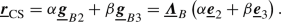(90)

Therein, $$\alpha \in {\mathbb {R}}^{}$$ and $$\beta \in {\mathbb {R}}^{}$$ are the beam cross-section coordinates, i.e. they parametrize the beam cross-section. In the following, two methods to enforce the 2D-3D coupling conditions (89) are presented, once with a Lagrange multiplier method and once with a quadratic penalty potential.

#### Penalty potential(91)

Here, $$\epsilon _{\text {2D-3D}}\in {\mathbb {R}}^{}$$ is a scalar penalty parameter. Variation of the penalty potential gives the following contributions to the weak form: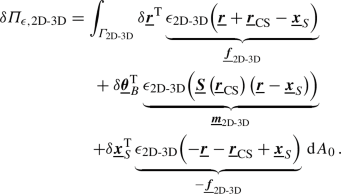(92)

Therein, the coupling force $$\underline{\varvec{{f}}}_\text {2D-3D}$$, acting on the beam centerline and solid, can be identified. Furthermore, $$\underline{\varvec{{m}}}_\text {2D-3D}$$ is the coupling moment acting on the beam cross-section. This demonstrates the projection of purely positional coupling constraints (on the surface of the beam) onto the beam centerline, and illustrates the arising rotational coupling terms in a 2D-3D coupling approach.

#### Lagrange multiplier potential

The 2D-3D coupling conditions (89) can also be enforced with a Lagrange multiplier method. A Lagrange multiplier vector field $$\underline{\varvec{{\lambda }}}_{\text {2D-3D}}\in {\mathbb {R}}^{3}$$ is therefore defined on the coupling surface $$\varGamma _{\text {2D-3D}}$$. The total Lagrange multiplier potential for the 2D-3D coupling reads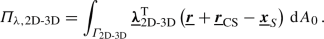(93)

The variation of the total Lagrange multiplier potential gives the following contributions to the weak form: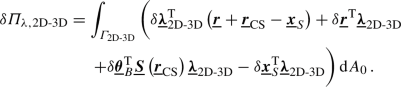(94)

Again, this showcases the projection onto the beam centerline, in this case of the Lagrange multiplier field $$\underline{\varvec{{\lambda }}}_{\text {2D-3D}}$$, i.e. the coupling surface tractions on the beam surface.

### Gauss point-to-segment approach for full 2D-3D coupling

Evaluating the variation of the total coupling potential (92) on the basis of the discretized solid position field and beam cross-section rotation field yields the discrete variation of the 2D-3D coupling potential: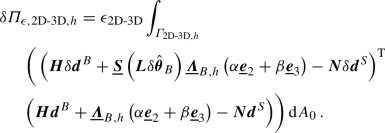(95)

Therein, $$\varGamma _{\text {2D-3D},h}$$ is the discrete beam surface. It is important to point out that the beam surface is not directly discretized. It is an analytical surface defined by the discretized beam centerline, the beam cross-section orientations and the beam cross-section geometry. Equation (95) can be stated in matrix form as

\begin{aligned}&\delta {\varPi _{\epsilon ,\text {2D-3D},h}}= \left[ \begin{array}{lll} {{\delta {{\varvec{{d}}^S}}}}^{\mathrm T}&{\delta \varvec{{d}}^B}^{\mathrm T}&{\delta \hat{\varvec{{\theta }}}_B}^{\mathrm T}\end{array}\right] \left[ \begin{array}{l} \int _{\varGamma _{\text {2D-3D},h}}{ \varvec{{f}}_{c,\text {2D-3D}}^{S} \,\mathrm d A_0}\, \\ \int _{\varGamma _{\text {2D-3D},h}}{ \varvec{{f}}_{c,r,\text {2D-3D}}^{B} \,\mathrm d A_0}\, \\ \int _{\varGamma _{\text {2D-3D},h}}{ \varvec{{f}}_{c,\theta ,\text {2D-3D}}^{B} \,\mathrm d A_0}\, \\ \end{array}\right] \nonumber \\&= \left[ \begin{array}{lll} {{\delta {{\varvec{{d}}^S}}}}^{\mathrm T}&{\delta \varvec{{d}}^B}^{\mathrm T}&{\delta \hat{\varvec{{\theta }}}_B}^{\mathrm T}\end{array}\right] \left[ \begin{array}{l} \varvec{{r}}_{c,\text {2D-3D}}^S\\ \varvec{{r}}_{c,r,\text {2D-3D}}^B\\ \varvec{{r}}_{c,\theta ,\text {2D-3D}}^B\\ \end{array}\right] , \end{aligned}
(96)

with the generalized point-wise 2D-3D coupling forces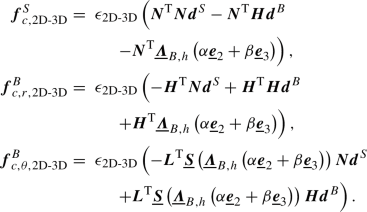(97)

Furthermore, $$\varvec{{r}}_{c,\text {2D-3D}}^S$$, $$\varvec{{r}}_{c,r,\text {2D-3D}}^B$$ and $$\varvec{{r}}_{c,\theta ,\text {2D-3D}}^B$$ are the local residual vectors. Again, a linearization of the residual contributions with respect to the discrete beam-to-solid pair degrees of freedom is required for the Newton–Raphson algorithm. The linearization reads: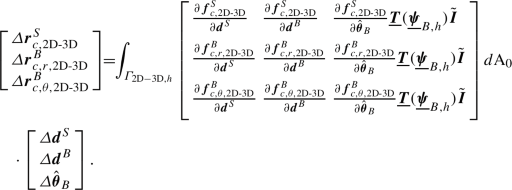(98)

The local contributions (96) and (98) to the global residual and the stiffness matrix, respectively, can be assembled in a straightforward manner and will not be stated here for the sake of brevity. As in the BTS-FULL mortar-type coupling, all derivatives explicitly stated in (98) are evaluated using forward automatic differentiation (FAD).

In practice, all integrals presented in this section are evaluated using a GPTS approach as illustrated in Figure 28, cf. . At each Gauss–Legendre point $${\tilde{\xi }}_i^B$$ along the beam centerline, multiple equally spaced coupling points (illustrated with the symbol ’$$\times$$’ in Figure 28) are defined along the circumference of the corresponding cross-section. Mechanically speaking, each coupling point is tied to the underlying solid via a linear penalty constraint.